# NCERT Exemplar Solutions for Class 9 Maths Chapter 10 - Circles

## NCERT Exemplar Solutions Class 9 Maths Chapter 10 – Free PDF Download

NCERT Exemplar Solutions for Class 9 Mathematics Chapter 10 Circles are provided here for students to prepare well for the final exams. Solving the exemplar questions will help them to understand the basics and advanced concepts of the chapter.  With the help of exemplar solutions, students can easily resolve their doubts while solving the exercise problems available in the NCERT textbook. These NCERT Exemplar Class 9 Maths solutions are designed by our subject experts with respect to the CBSE syllabus (2023-2024) for Chapter 10 based on the topics given below:

• Circles and their related terms like arc, sector, chords, semicircle, segments, etc.
• The angle subtended by chords of a circle at a point and theorems based on it
• The perpendicular from the Centre to a Chord and theorems based on it
• Circle through three points and its related theorems
• Chords equidistant from the centre of a circle
• Angle subtended by an arc of a circle
• Angles in the same segment of a circle
• Cyclic quadrilaterals and related theorems

Exemplar Solutions for Chapter 10, Circles, can be used as a reference tool for quick revision by students of Class 9 during the exams. They can also use other study materials available on our websites, such as NCERT Solutions, exemplar books, notes, and previous years’ question papers to prepare for the Maths exam. Learn and score good marks with the help of these learning materials, which follow the NCERT curriculum. Also, solve sample papers and previous years’ question papers to get a clear idea of the types of questions asked from Chapter 10.

## Download the PDF of NCERT Exemplar Solutions for Class 9 Maths Chapter 10 Circles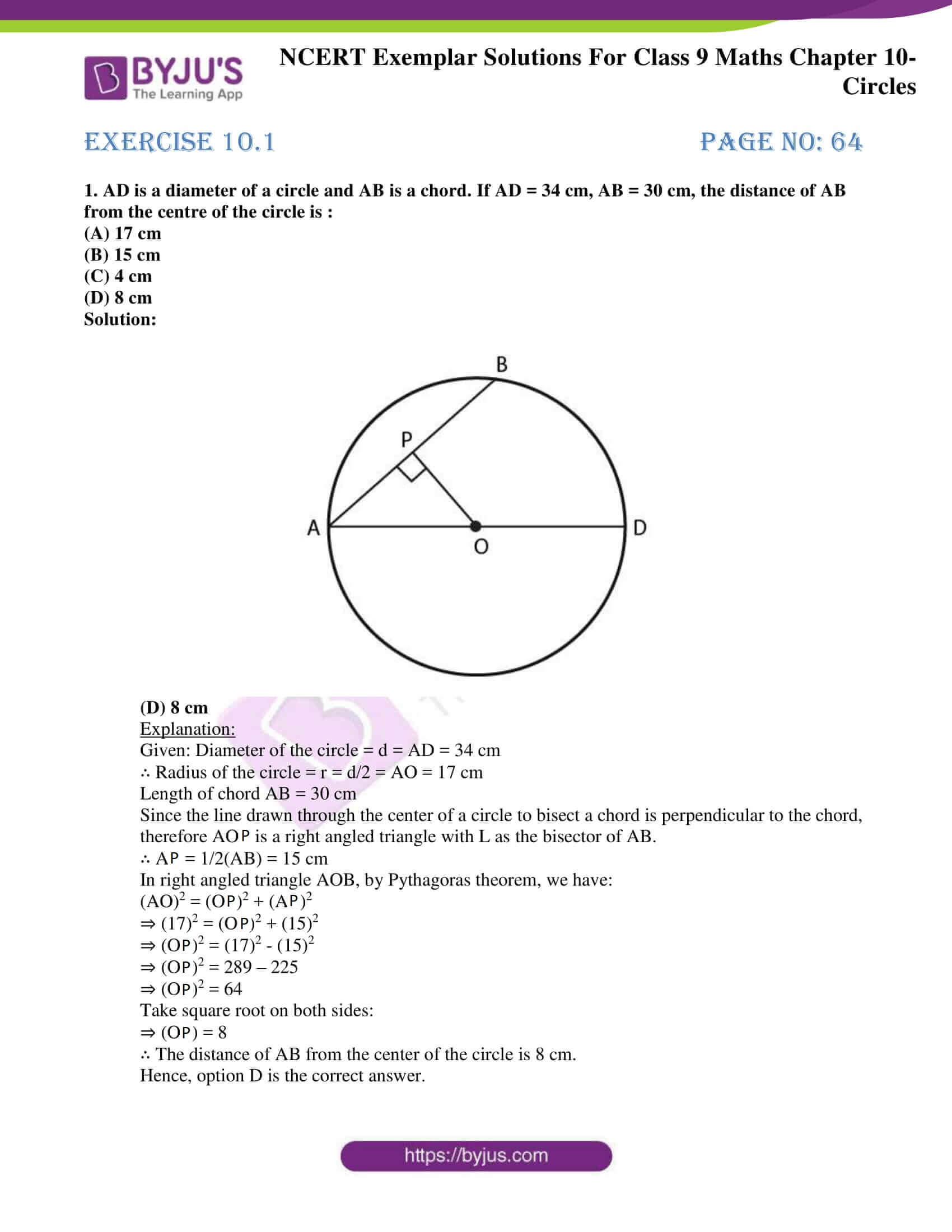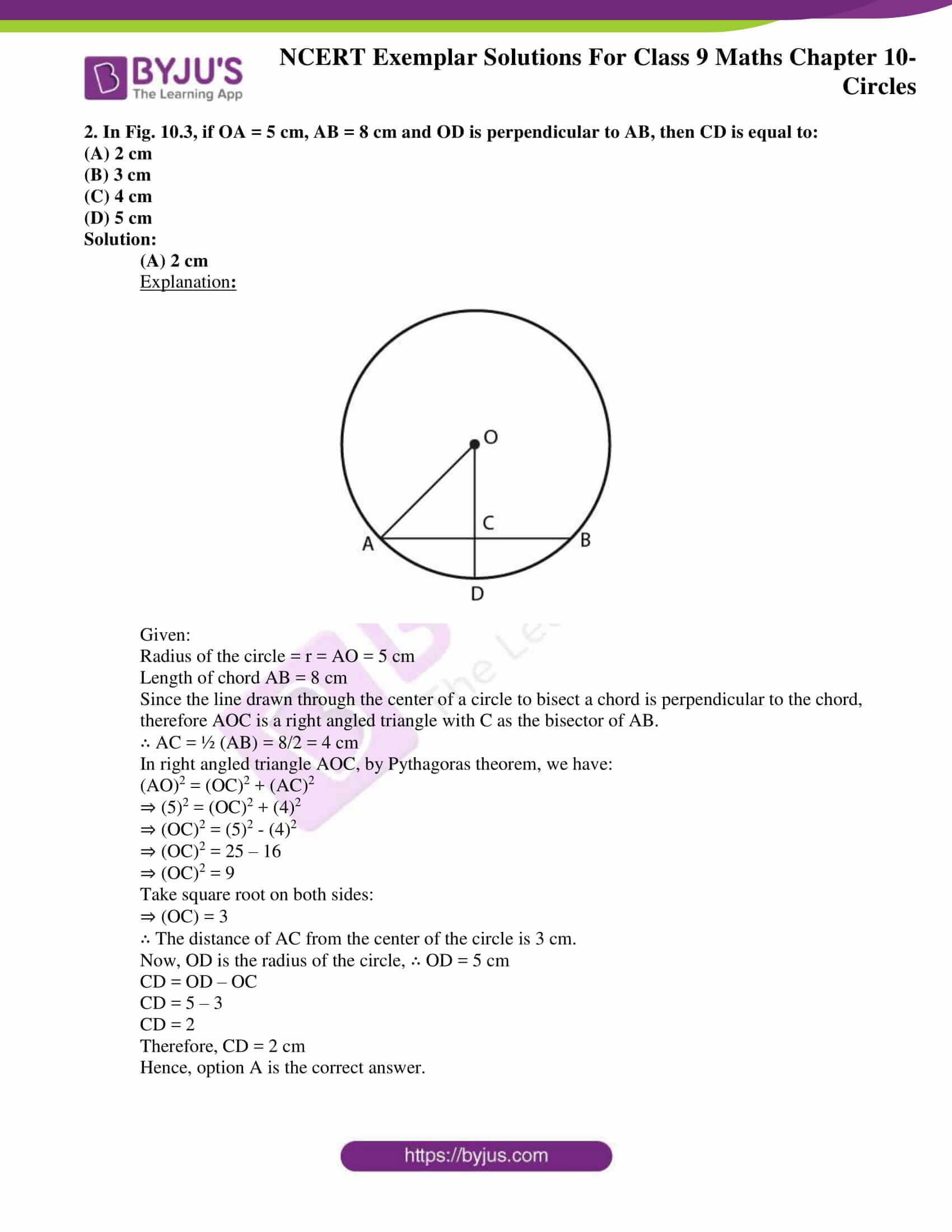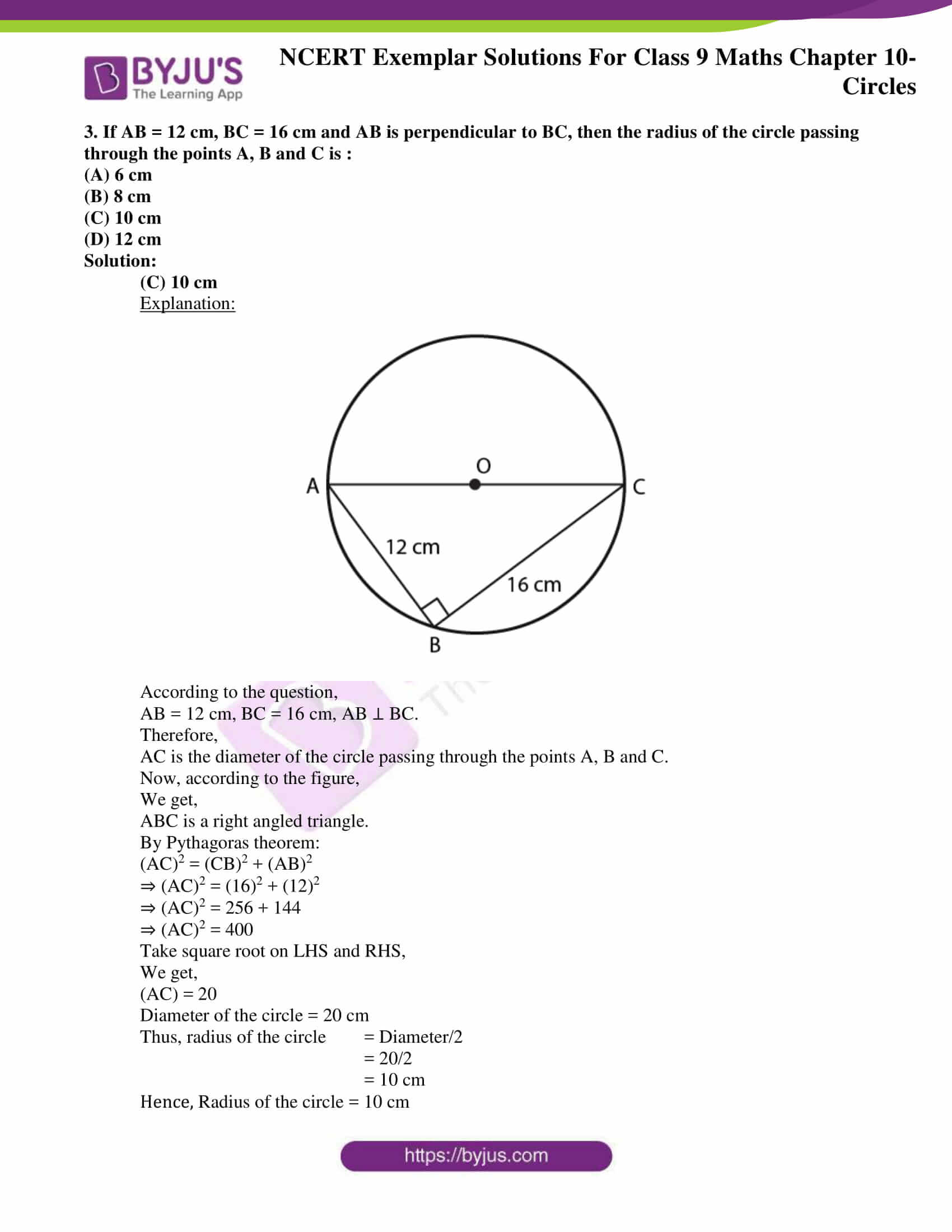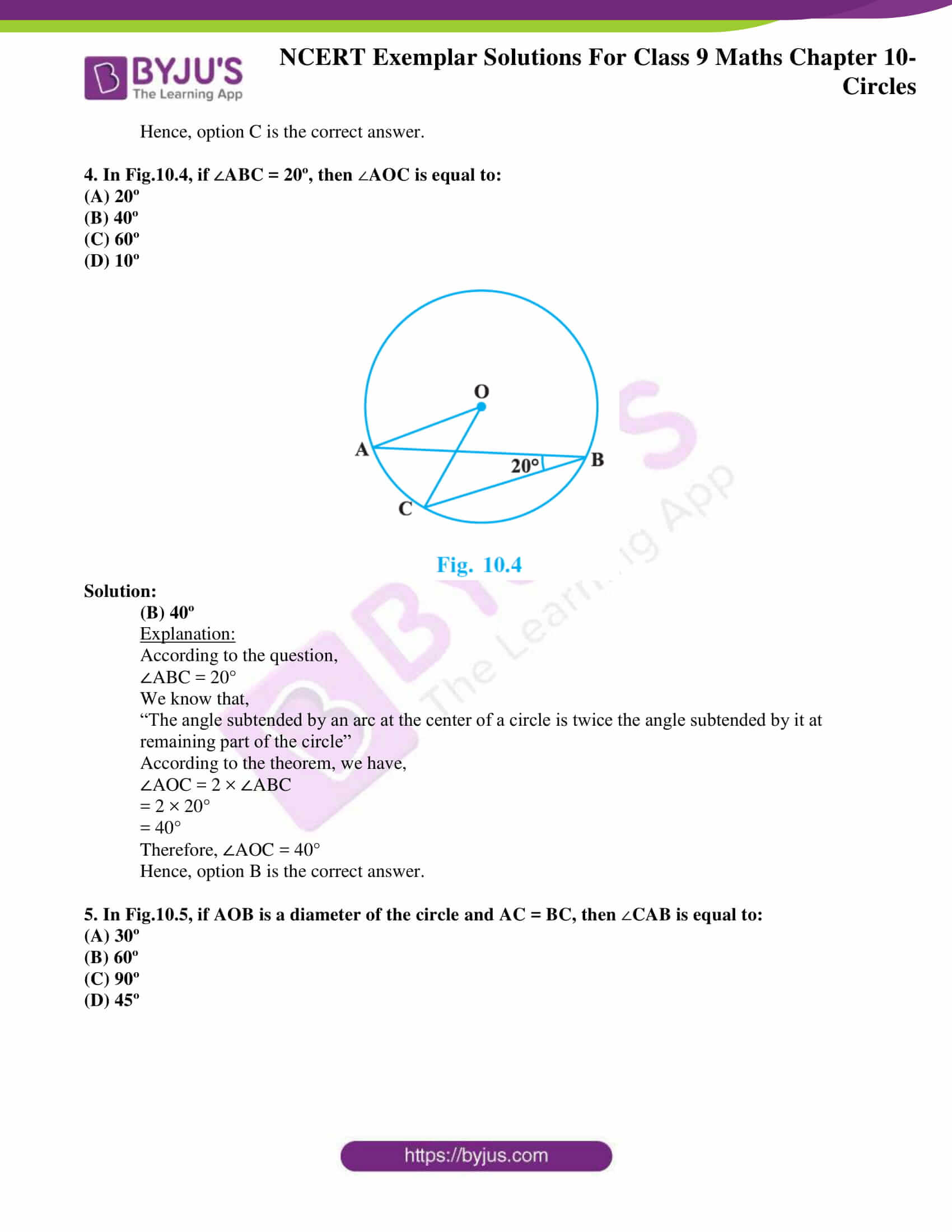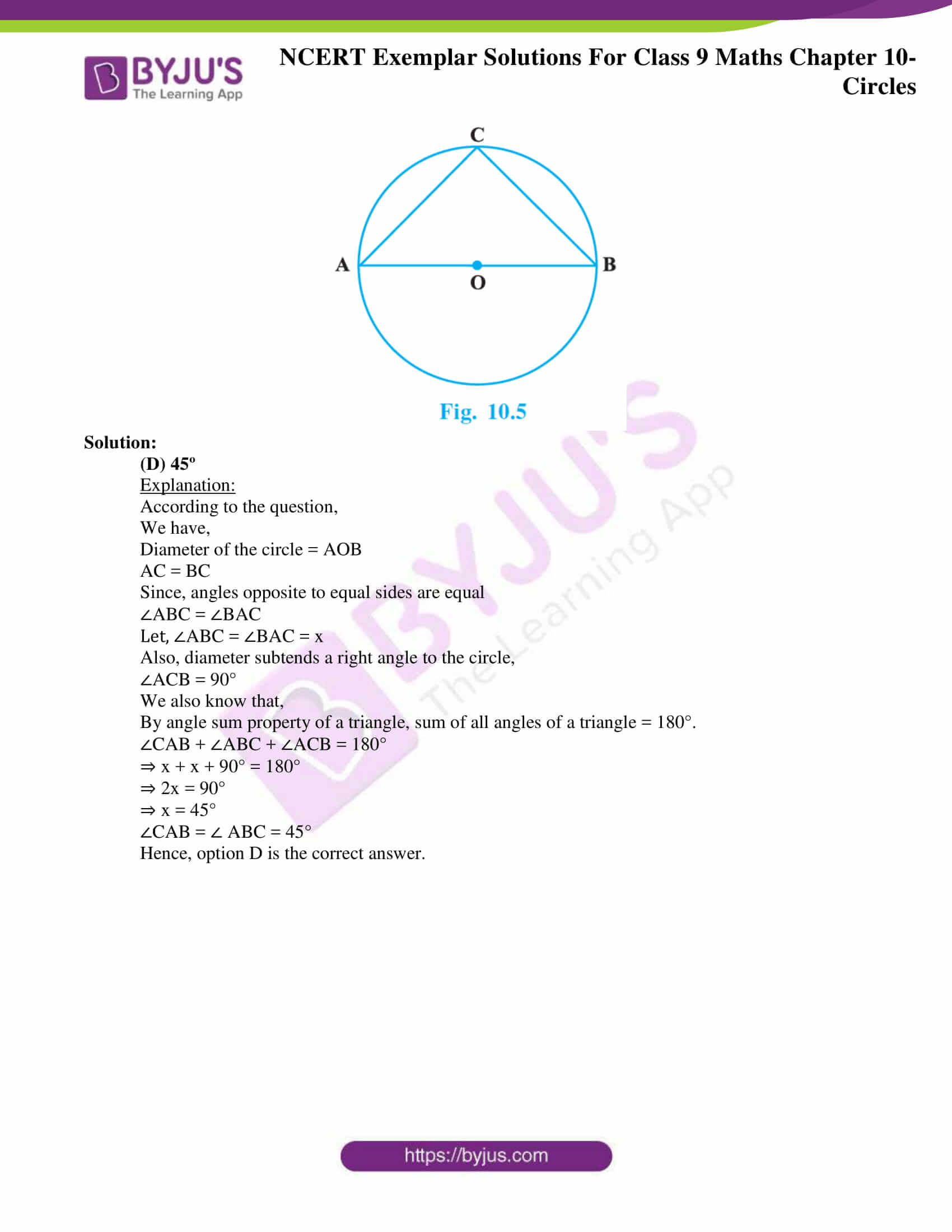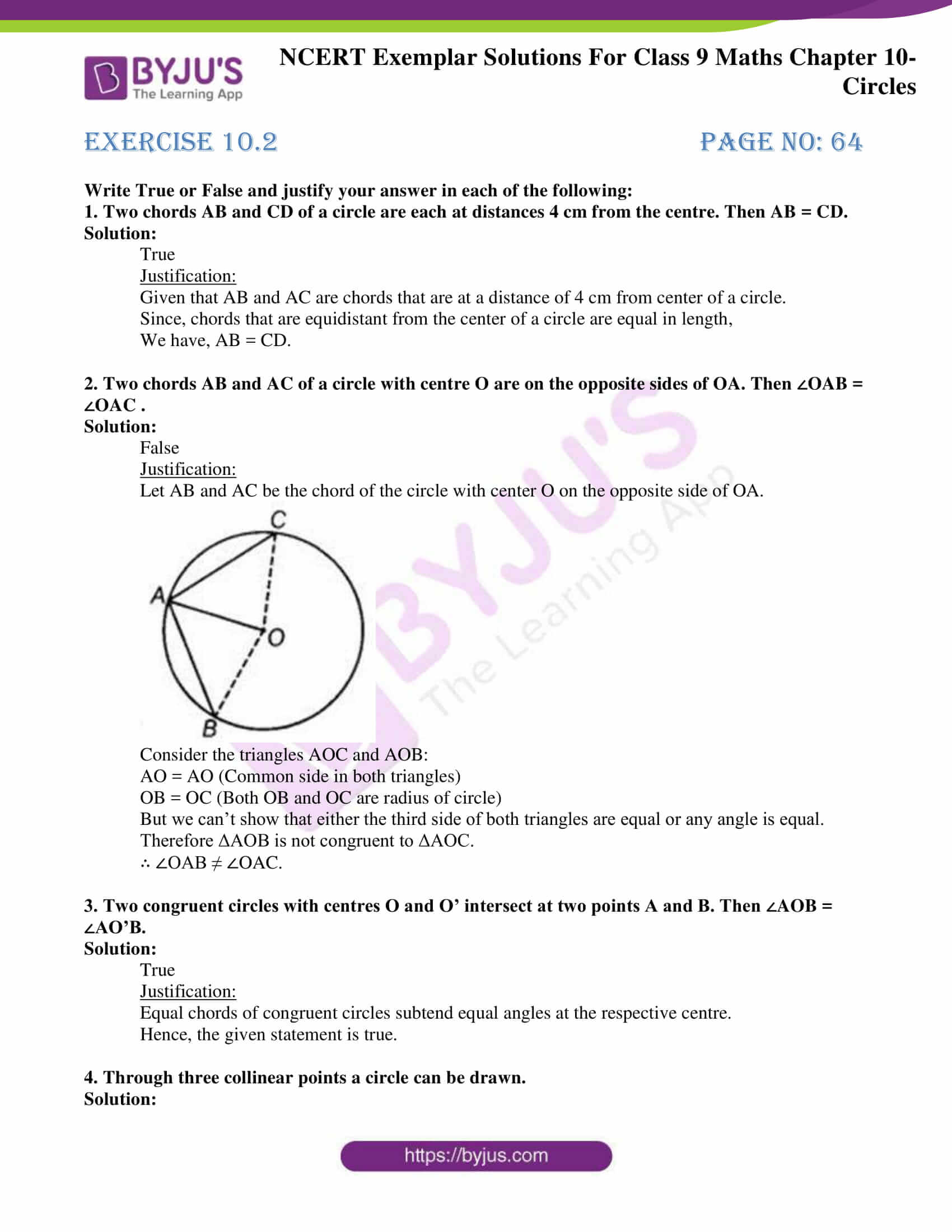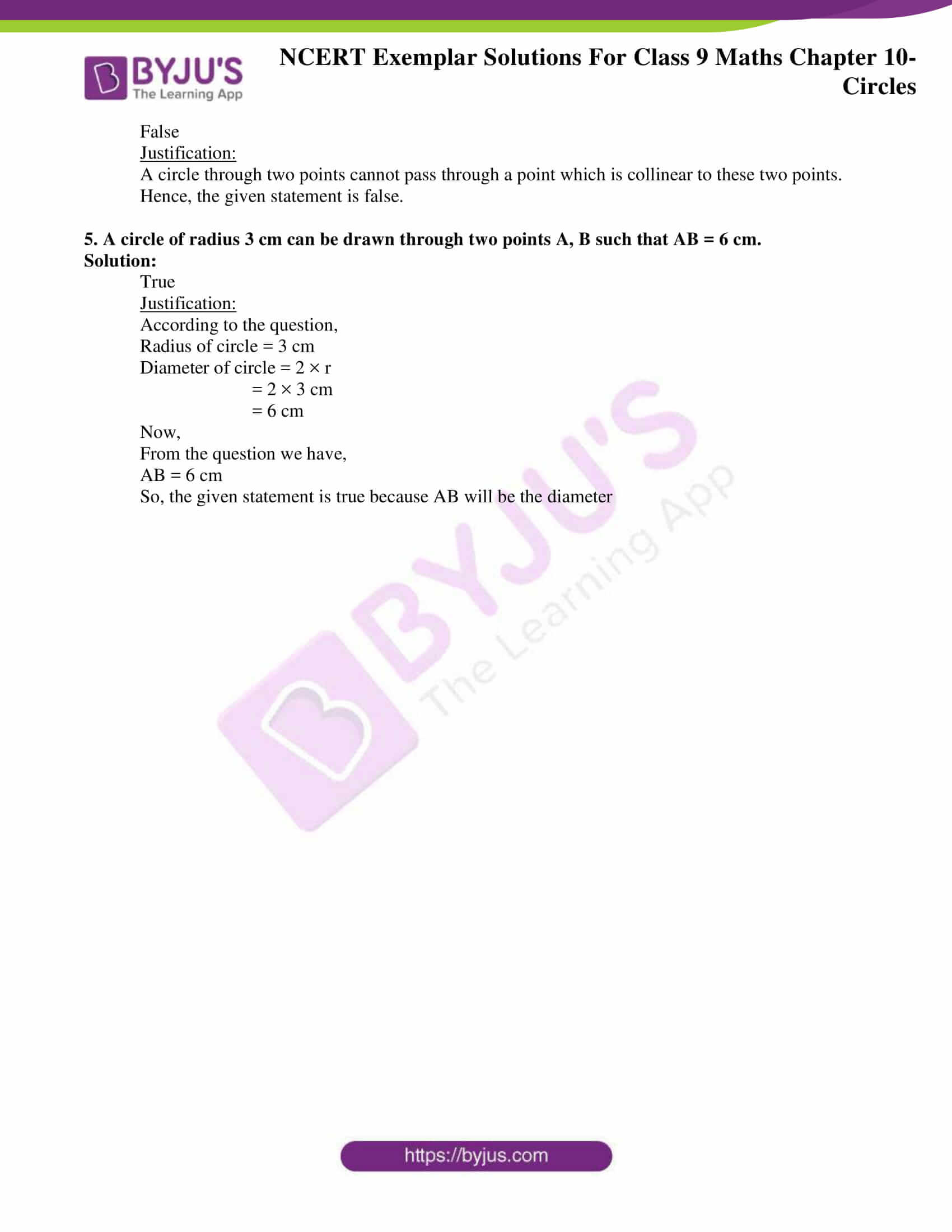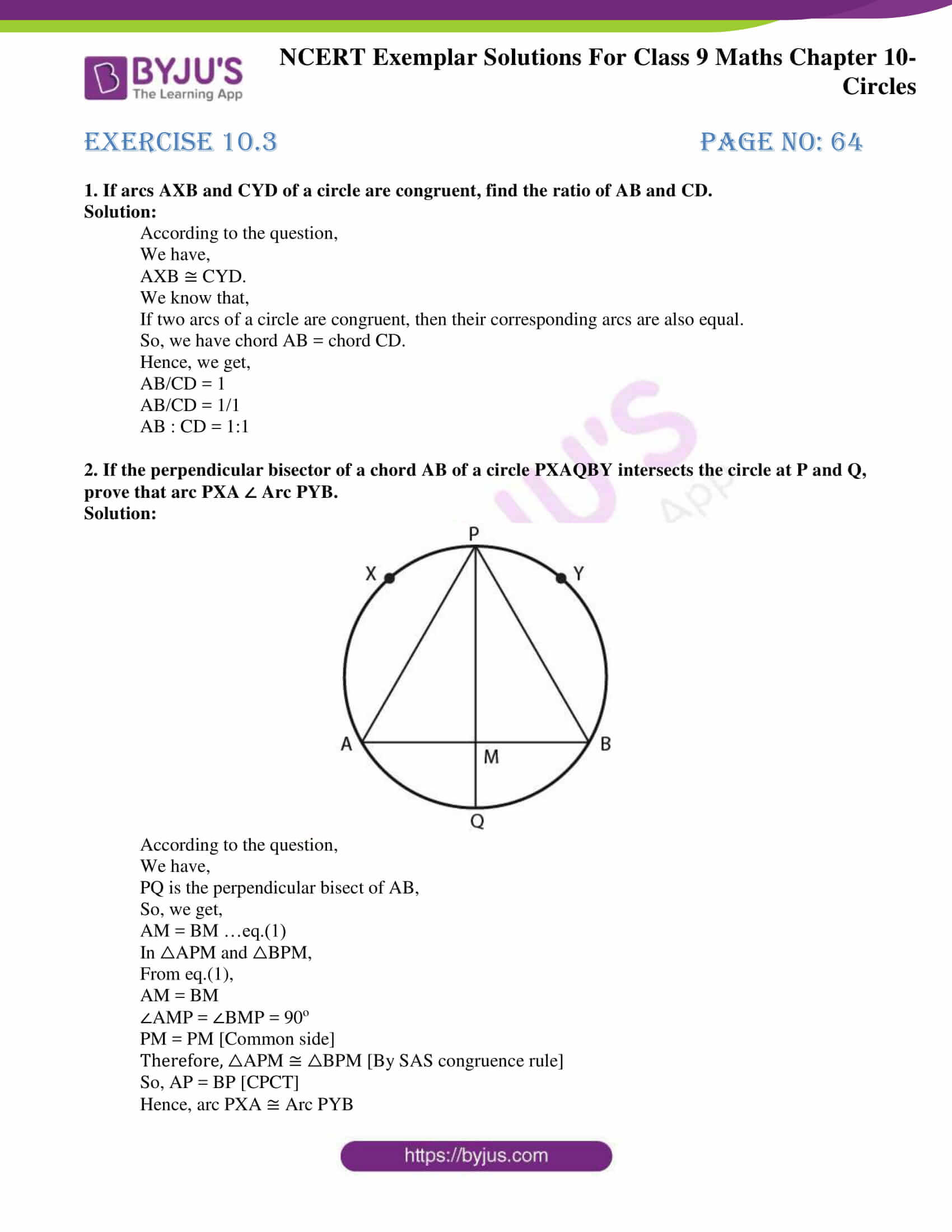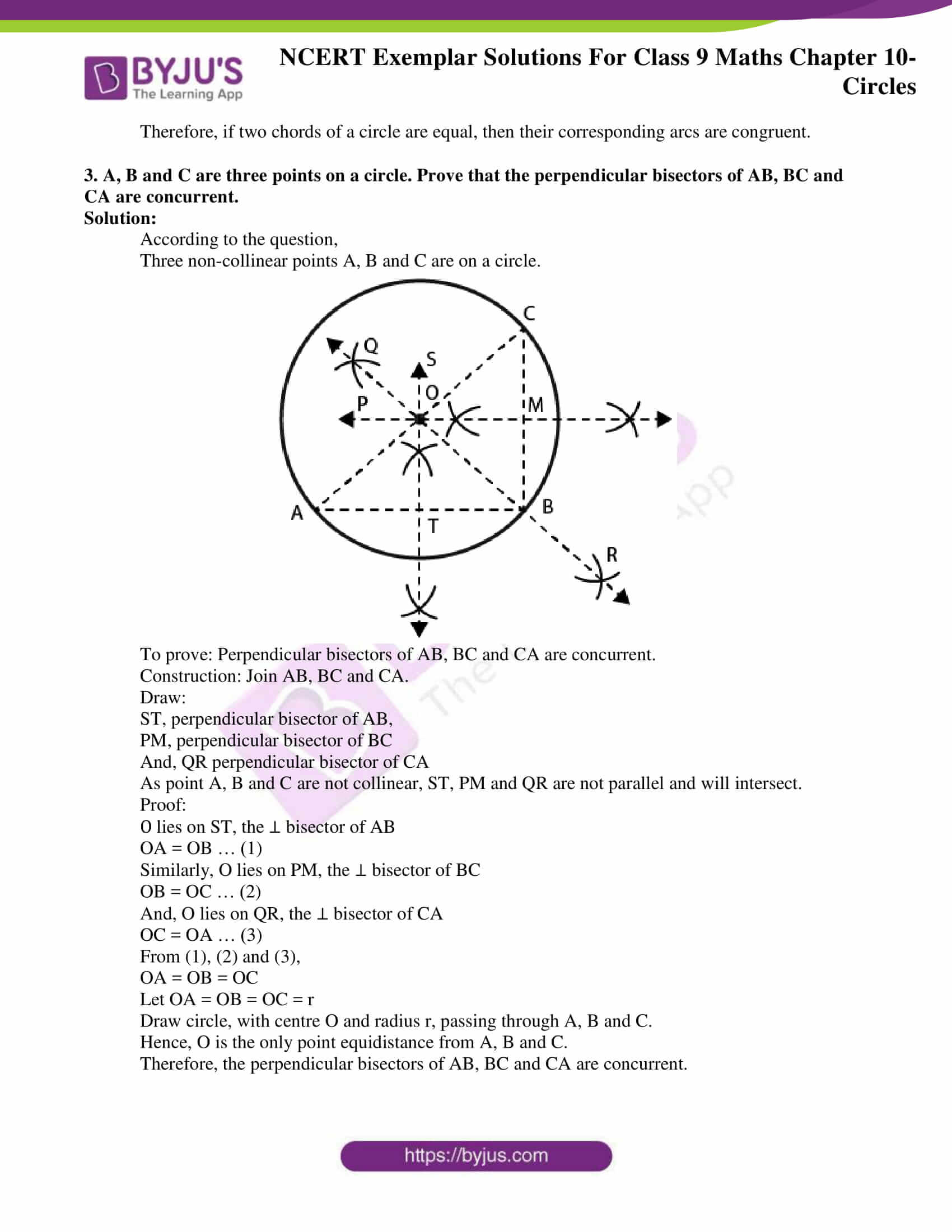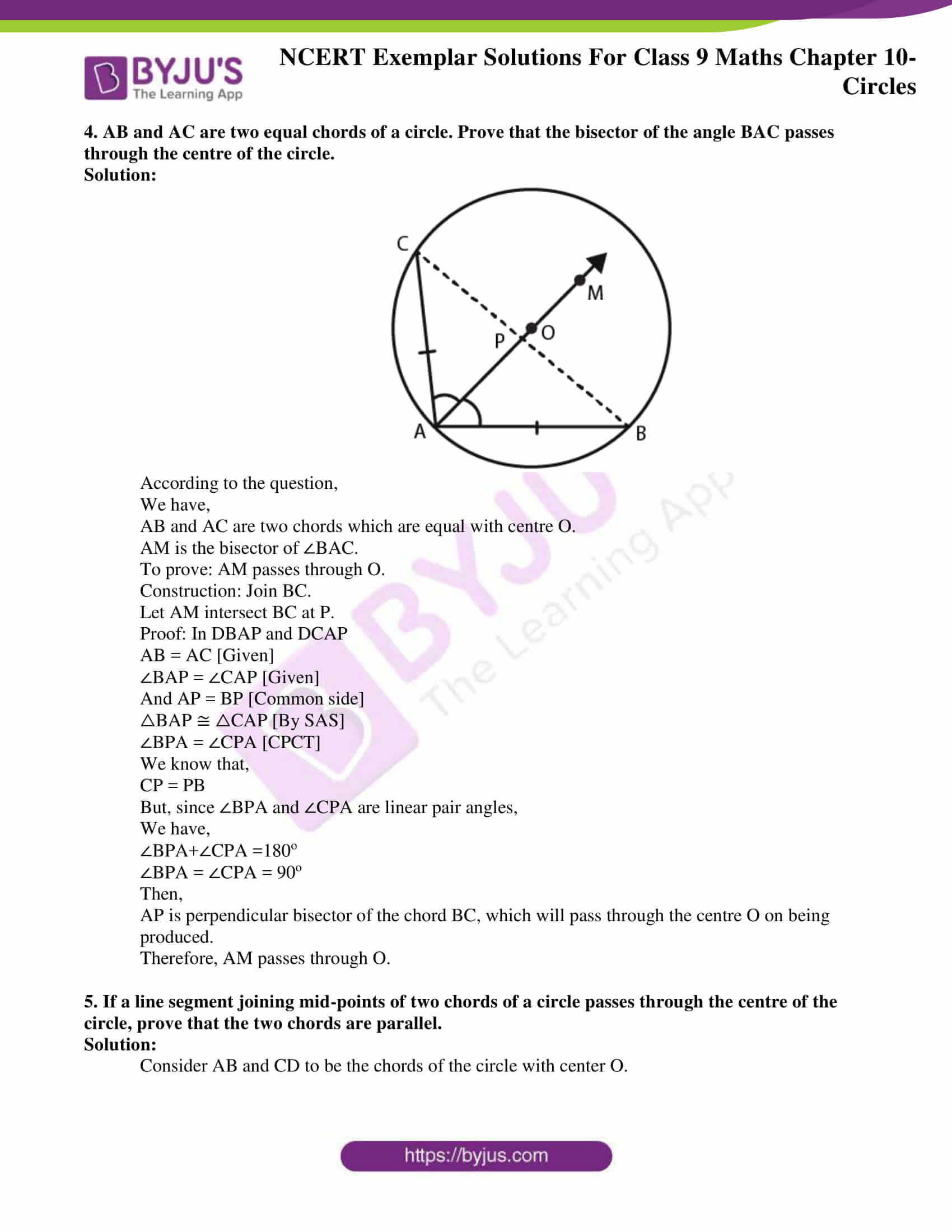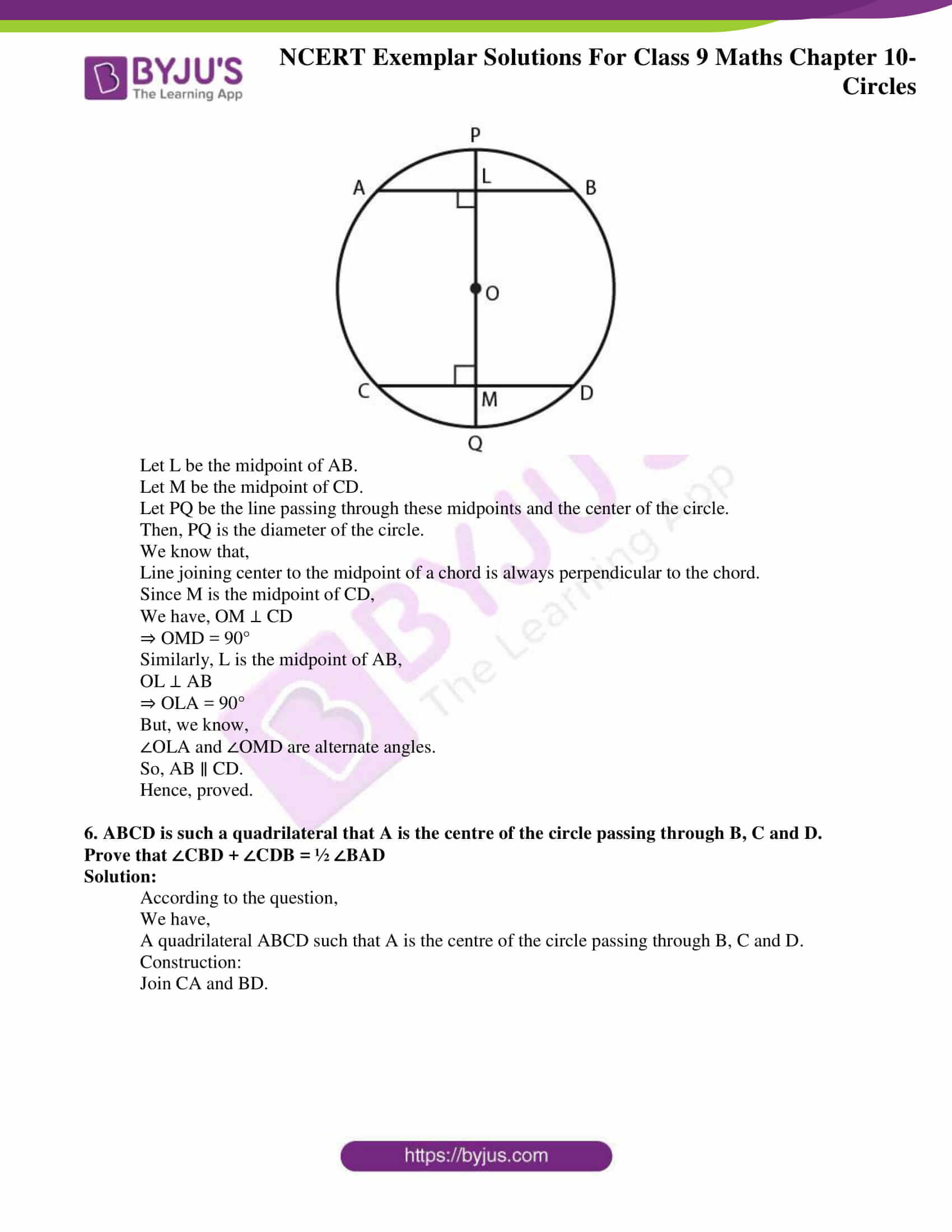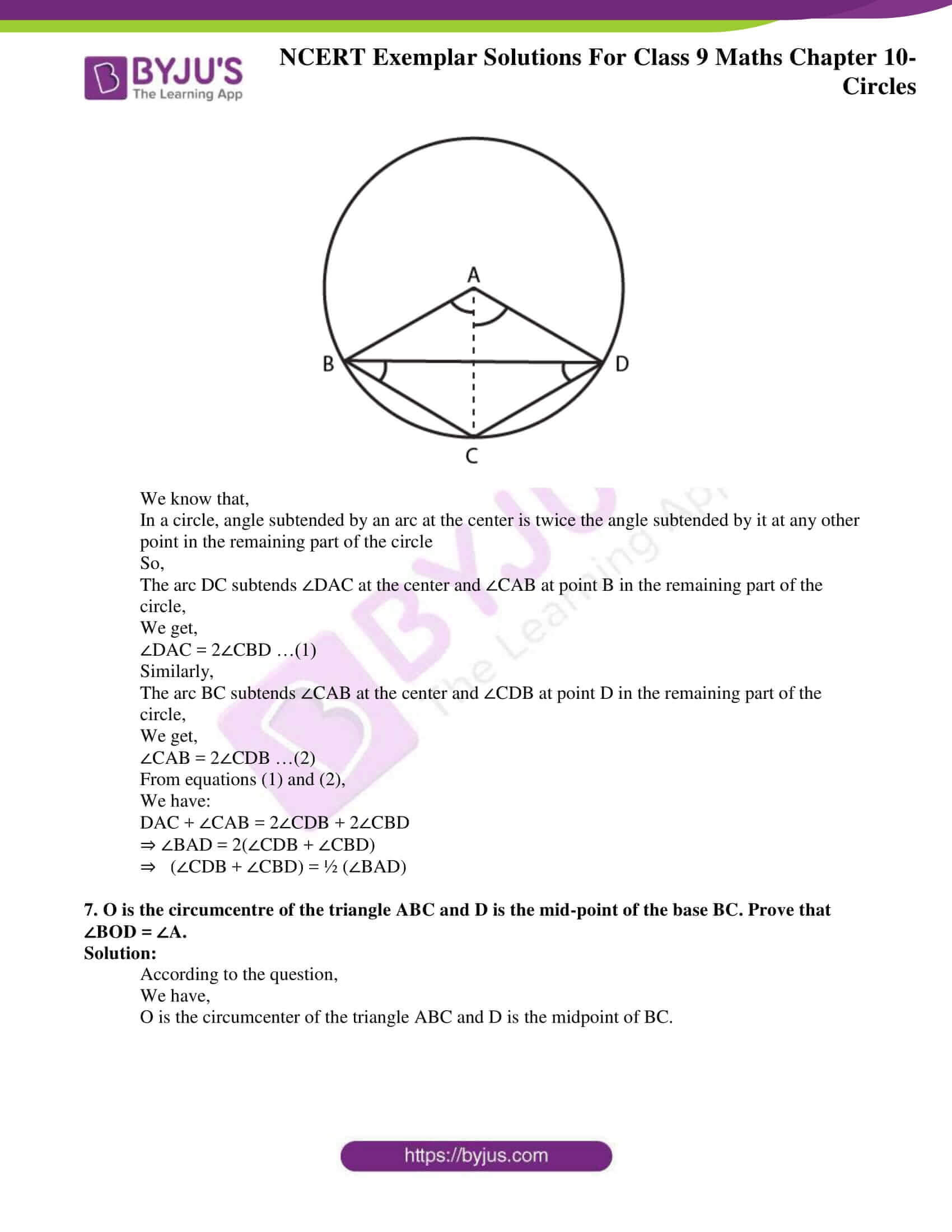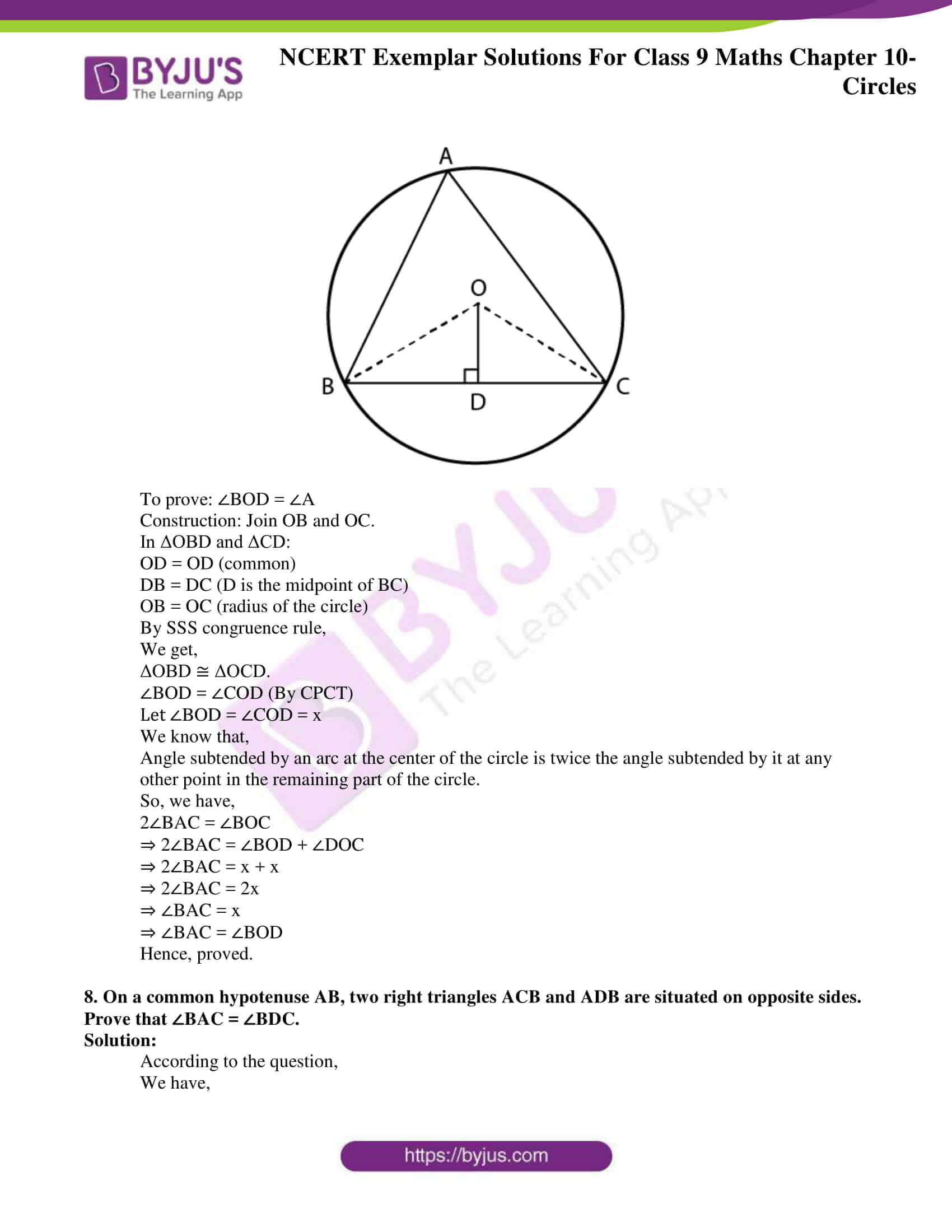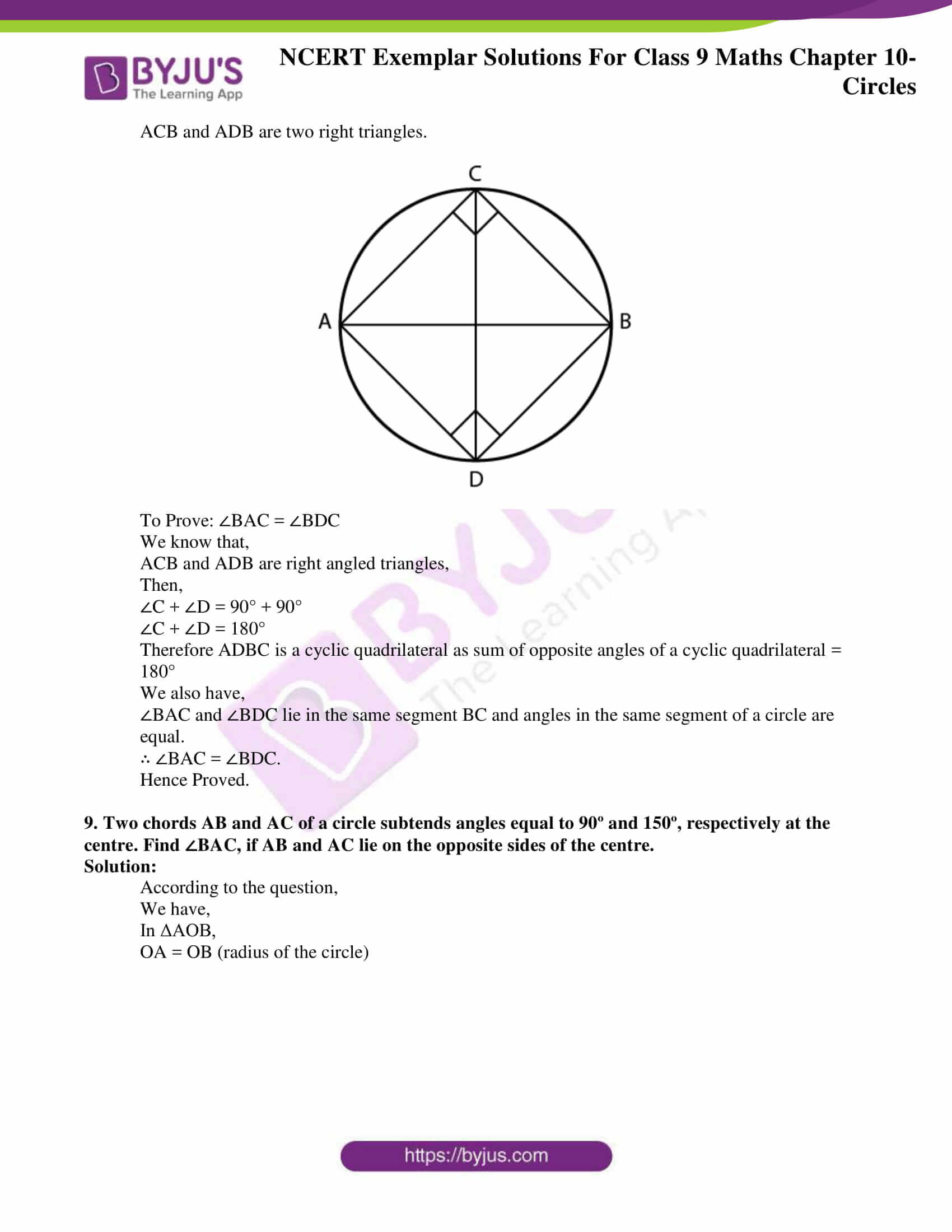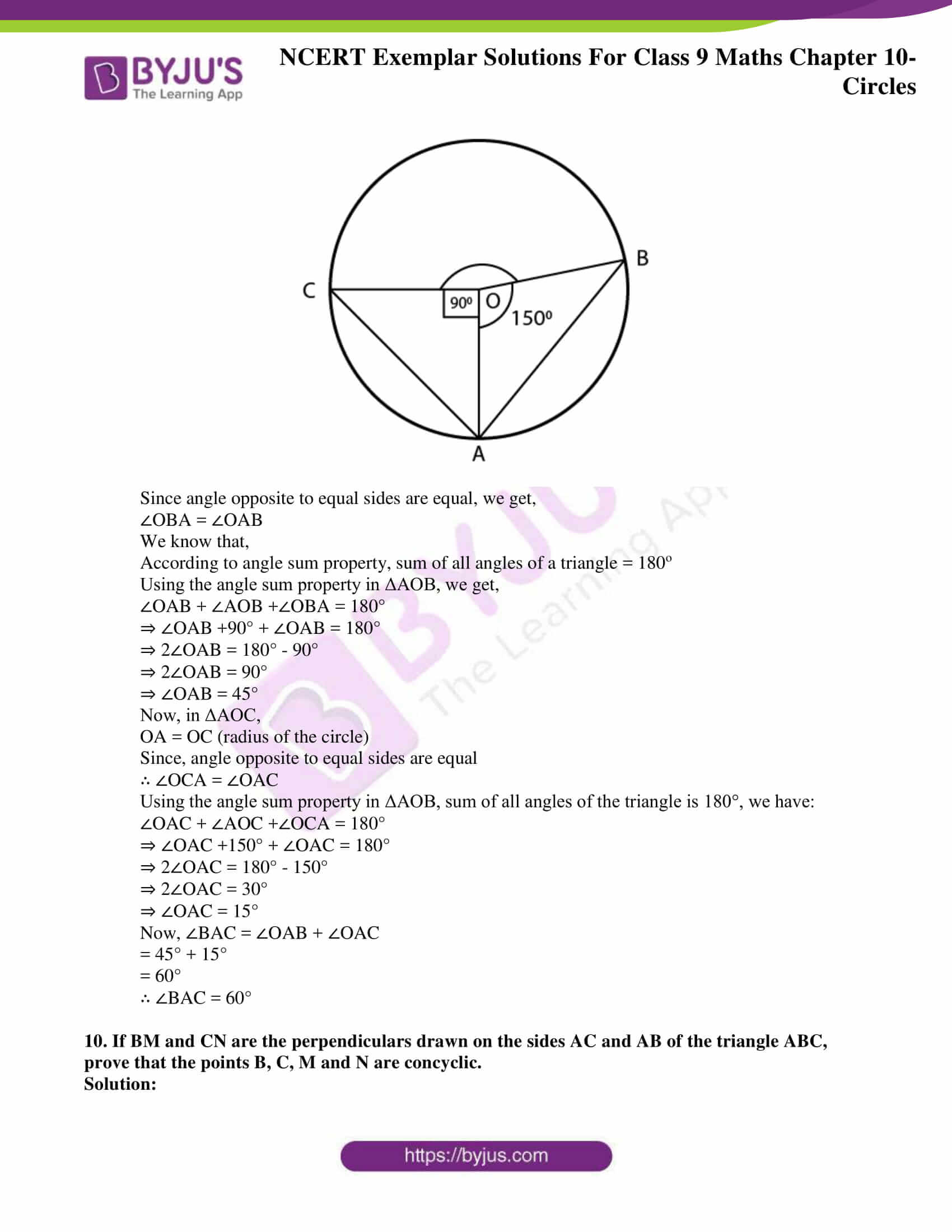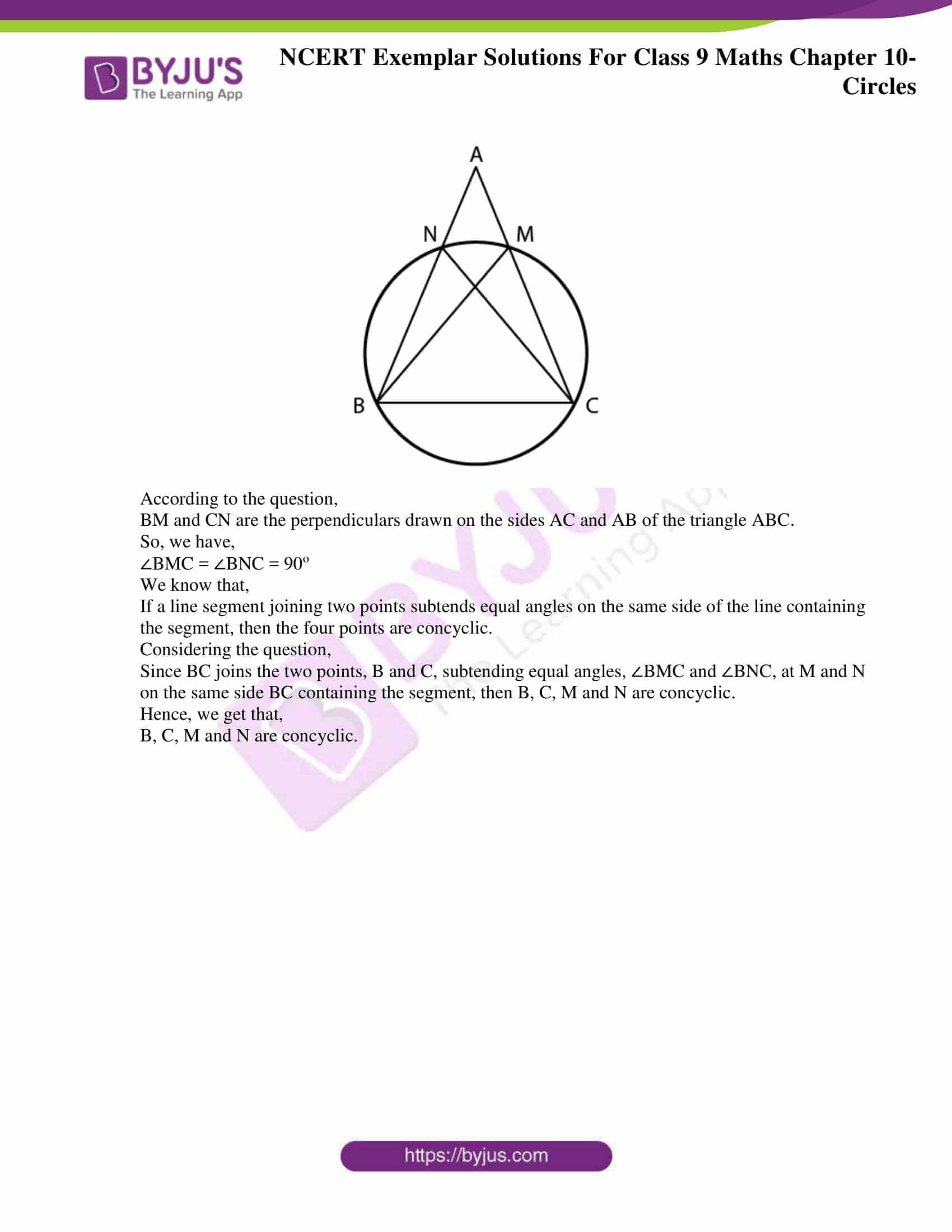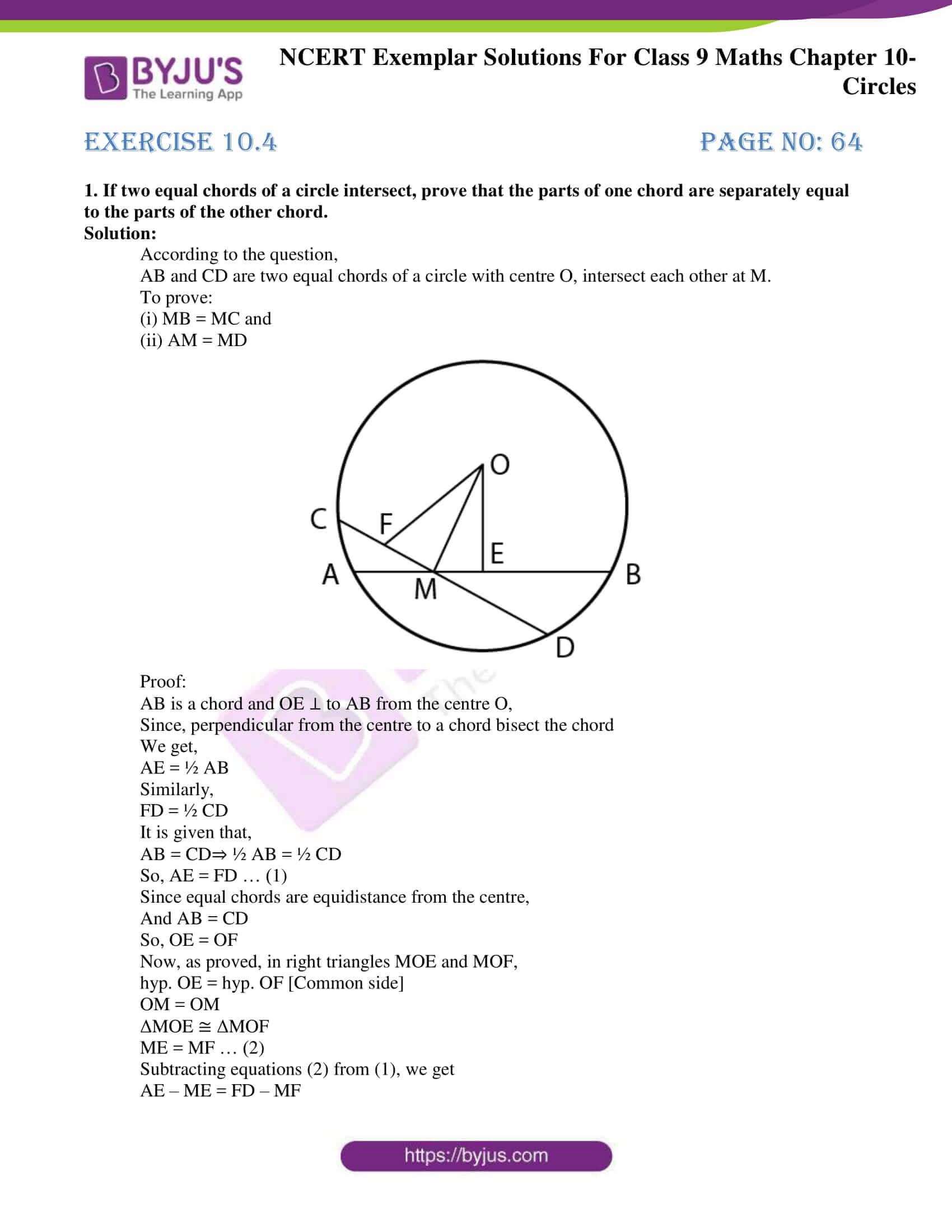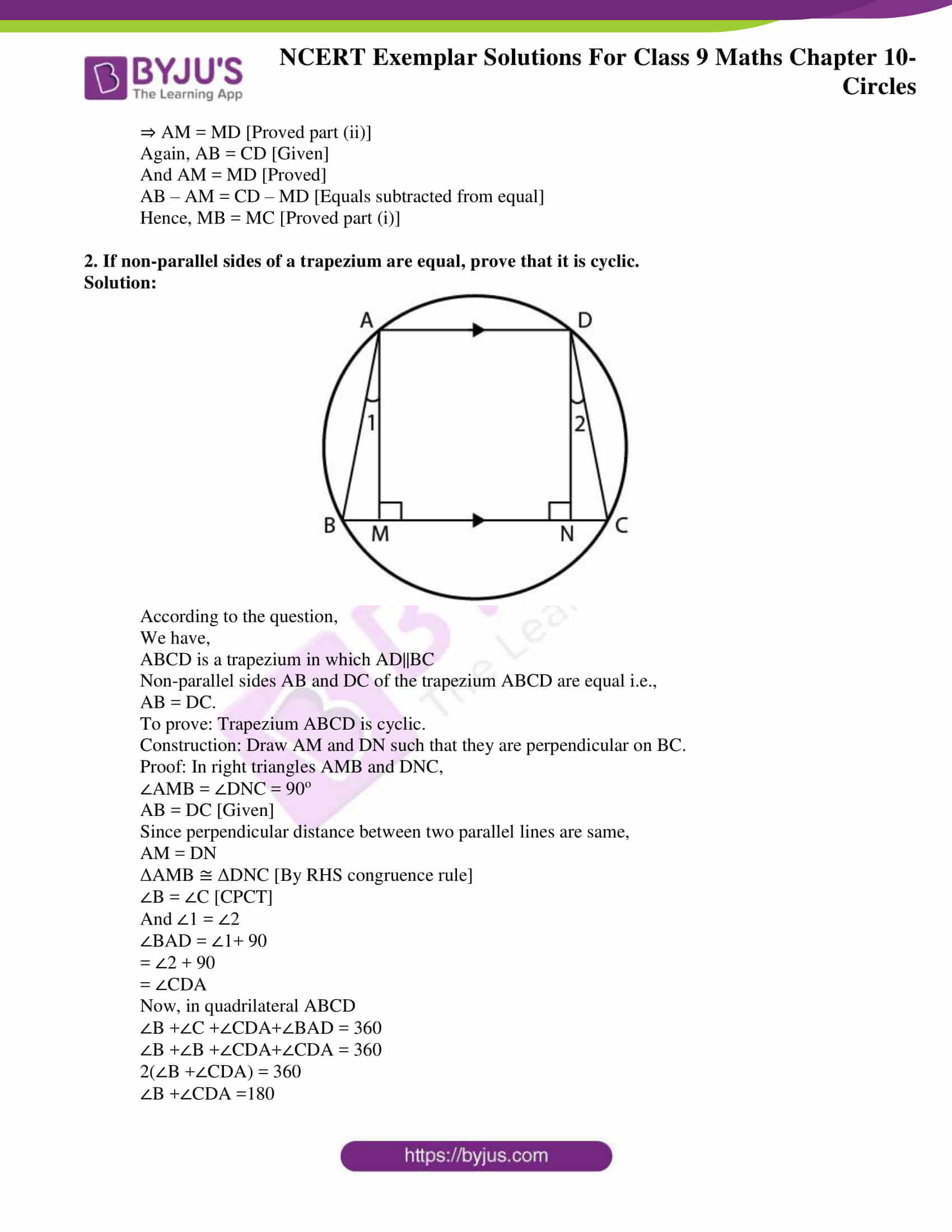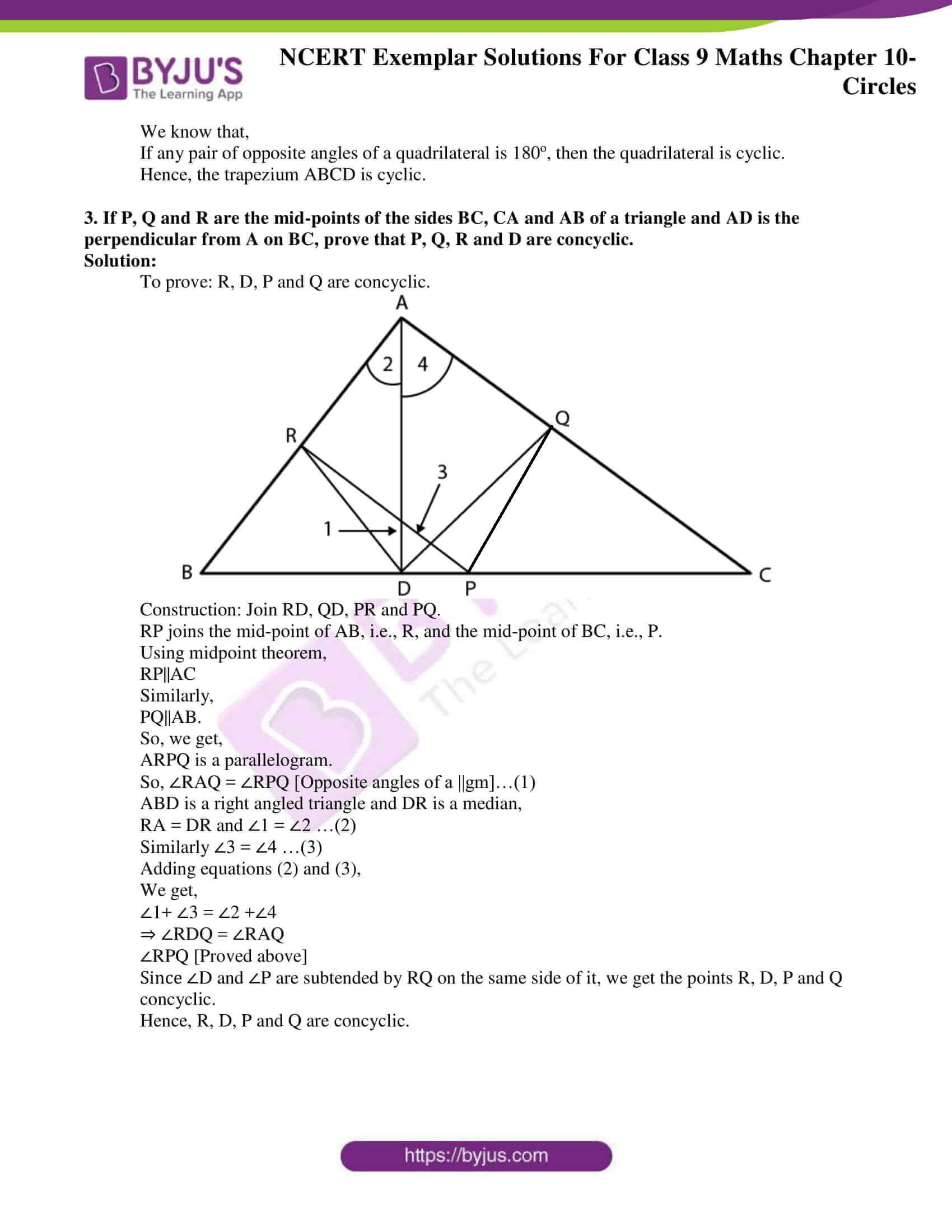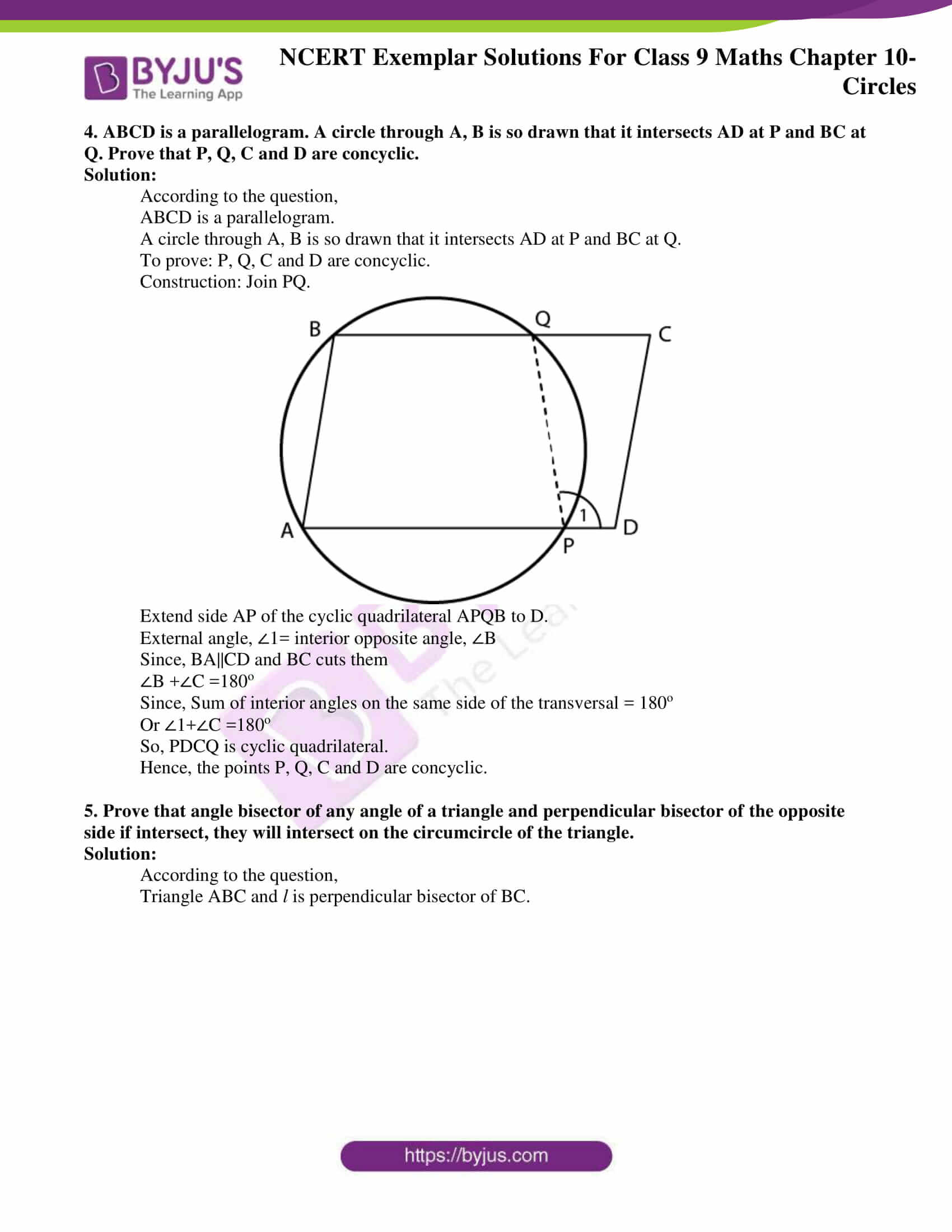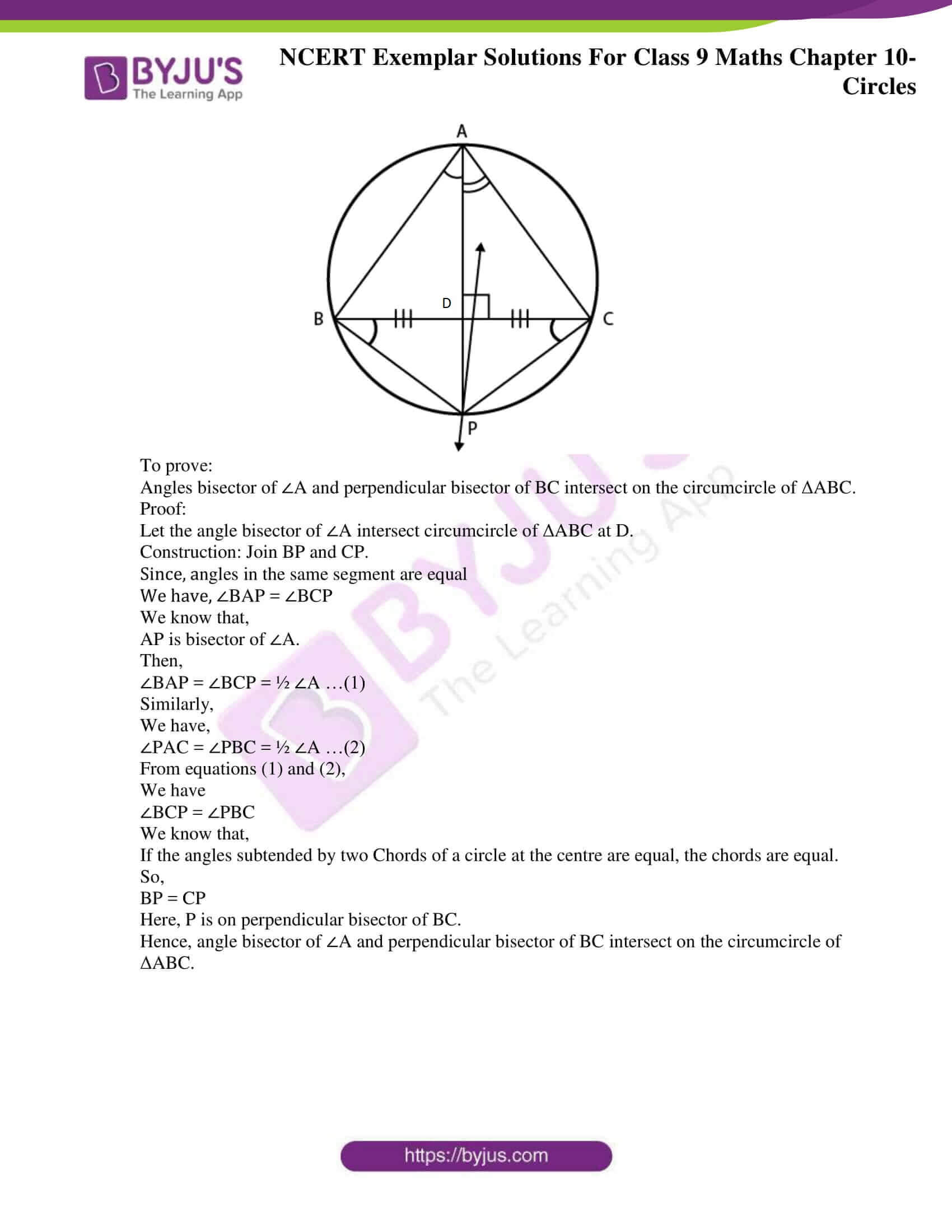### Access Answers to NCERT Exemplar Solutions for Class 9 Maths Chapter 10 Circles

Exercise 10.1 Page No: 64

1. AD is a diameter of a circle and AB is a chord. If AD = 34 cm, AB = 30 cm, the distance of AB from the centre of the circle is :

(A) 17 cm

(B) 15 cm

(C) 4 cm

(D) 8 cm

Solution: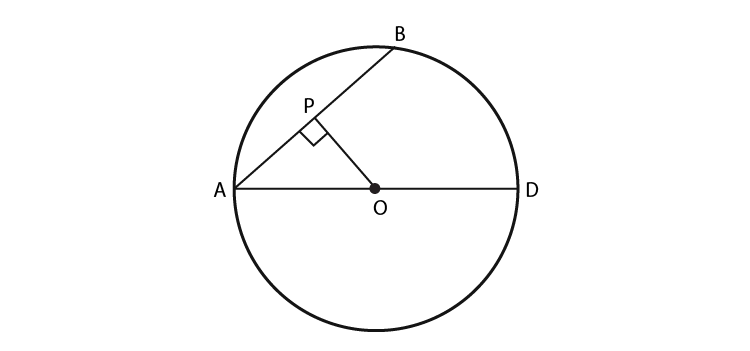(D) 8 cm

Explanation:

Given: Diameter of the circle = d = AD = 34 cm

∴ Radius of the circle = r = d/2 = AO = 17 cm

Length of chord AB = 30 cm

Since the line drawn through the centre of a circle to bisect a chord is perpendicular to the chord, therefore AOP is a right angled triangle with L as the bisector of AB.

∴ AP = 1/2(AB) = 15 cm

In right angled triangle AOB, by Pythagoras theorem, we have:

(AO)2 = (OP)2 + (AP)2

⇒ (17)2 = (OP)2 + (15)2

⇒ (OP)2 = (17)2 – (15)2

⇒ (OP)2 = 289 – 225

⇒ (OP)2 = 64

Take square root on both sides:

⇒ (OP) = 8

∴ The distance of AB from the centre of the circle is 8 cm.

Hence, option D is the correct answer.

2. In Fig. 10.3, if OA = 5 cm, AB = 8 cm and OD is perpendicular to AB, then CD is equal to:

(A) 2 cm

(B) 3 cm

(C) 4 cm

(D) 5 cm

Solution:

(A) 2 cm

Explanation: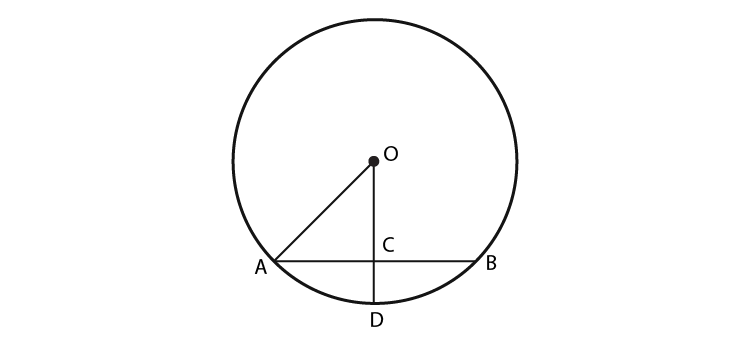Given:

Radius of the circle = r = AO = 5 cm

Length of chord AB = 8 cm

Since the line drawn through the centre of a circle to bisect a chord is perpendicular to the chord, therefore AOC is a right angled triangle with C as the bisector of AB.

∴ AC = ½ (AB) = 8/2 = 4 cm

In right angled triangle AOC, by Pythagoras theorem, we have:

(AO)2 = (OC)2 + (AC)2

⇒ (5)2 = (OC)2 + (4)2

⇒ (OC)2 = (5)2 – (4)2

⇒ (OC)2 = 25 – 16

⇒ (OC)2 = 9

Take square root on both sides:

⇒ (OC) = 3

∴ The distance of AC from the centre of the circle is 3 cm.

Now, OD is the radius of the circle, ∴ OD = 5 cm

CD = OD – OC

CD = 5 – 3

CD = 2

Therefore, CD = 2 cm

Hence, option A is the correct answer.

3. If AB = 12 cm, BC = 16 cm and AB is perpendicular to BC, then the radius of the circle passing through the points A, B and C is :

(A) 6 cm

(B) 8 cm

(C) 10 cm

(D) 12 cm

Solution:

(C) 10 cm

Explanation: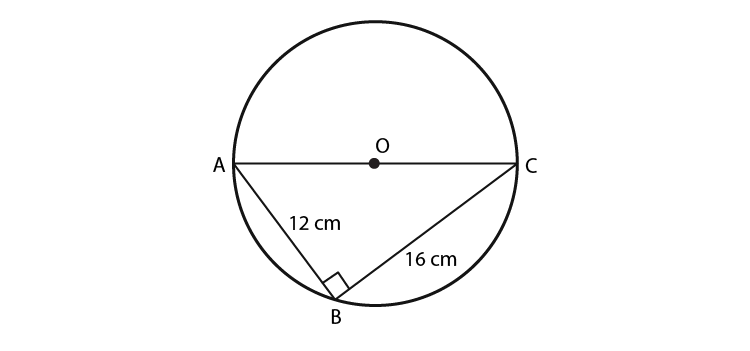According to the question,

AB = 12 cm, BC = 16 cm, AB ⊥ BC.

Therefore,

AC is the diameter of the circle passing through the points A, B and C.

Now, according to the figure,

We get,

ABC is a right angled triangle.

By Pythagoras theorem:

(AC)2 = (CB)2 + (AB)2

⇒ (AC)2 = (16)2 + (12)2

⇒ (AC)2 = 256 + 144

⇒ (AC)2 = 400

Take square root on LHS and RHS,

We get,

(AC) = 20

Diameter of the circle = 20 cm

Thus, the radius of the circle = Diameter/2

= 20/2

= 10 cm

Hence, the radius of the circle = 10 cm

Hence, option C is the correct answer.

4. In Fig.10.4, if ∠ABC = 20º, then AOC is equal to:

(A) 20º

(B) 40º

(C) 60º

(D) 10º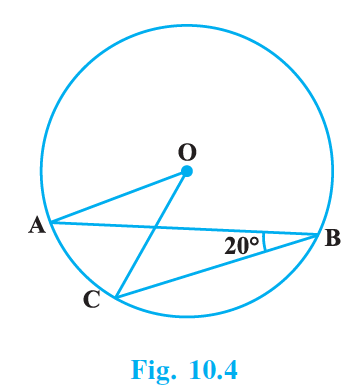Solution:

(B) 40º

Explanation:

According to the question,

∠ABC = 20°

We know that,

“The angle subtended by an arc at the centre of a circle is twice the angle subtended by it at the remaining part of the circle.”

According to the theorem, we have,

∠AOC = 2 × ∠ABC

= 2 × 20°

= 40°

Therefore, ∠AOC = 40°

Hence, option B is the correct answer.

5. In Fig.10.5, if AOB is a diameter of the circle and AC = BC, then CAB is equal to:

(A) 30º

(B) 60º

(C) 90º

(D) 45º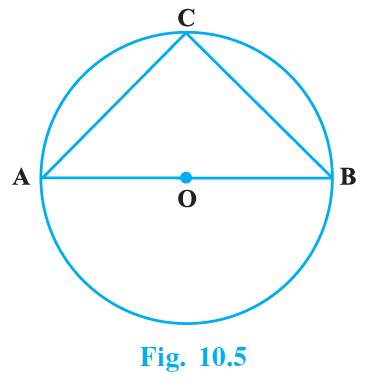Solution:

(D) 45º

Explanation:

According to the question,

We have,

Diameter of the circle = AOB

AC = BC

Since, angles opposite to equal sides are equal

∠ABC = ∠BAC

Let, ∠ABC = ∠BAC = x

Also, diameter subtends a right angle to the circle,

∠ACB = 90°

We also know that,

By angle sum property of a triangle, sum of all angles of a triangle = 180°.

∠CAB + ∠ABC + ∠ACB = 180°

⇒ x + x + 90° = 180°

⇒ 2x = 90°

⇒ x = 45°

∠CAB = ∠ ABC = 45°

Hence, option D is the correct answer.

Exercise 10.2 Page No: 64

Write True or False and justify your answer in each of the following:

1. Two chords AB and CD of a circle are each at distances 4 cm from the centre. Then AB = CD.

Solution:

True

Justification:

Given that AB and AC are chords that are at a distance of 4 cm from the centre of a circle.

Since, chords that are equidistant from the centre of a circle are equal in length,

We have, AB = CD.

2. Two chords AB and AC, of a circle with centre O are on the opposite sides of OA. Then ∠OAB = ∠OAC.

Solution:

False

Justification:

Let AB and AC be the chord of the circle with centre O on the opposite side of OA.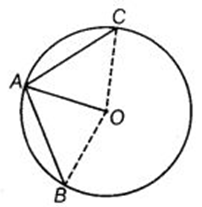Consider the triangles AOC and AOB:

AO = AO (Common side in both triangles)

OB = OC (Both OB and OC are radius of the circle)

But we can’t show that either the third side of both triangles is equal or any angle is equal. Therefore ΔAOB is not congruent to ΔAOC.

∴ ∠OAB ≠ ∠OAC.

3. Two congruent circles with centres O and O’ intersect at two points A and B. Then ∠AOB = ∠AO’B.

Solution:

True

Justification:

Equal chords of congruent circles subtend equal angles at the respective centre.

Hence, the given statement is true.

4. Through three collinear points a circle can be drawn.

Solution:

False

Justification:

A circle through two points cannot pass through a point which is collinear to these two points.

Hence, the given statement is false.

5. A circle of radius 3 cm can be drawn through two points A, B such that AB = 6 cm.

Solution:

True

Justification:

According to the question,

Radius of circle = 3 cm

Diameter of circle = 2 × r

= 2 × 3 cm

= 6 cm

Now,

From the question we have,

AB = 6 cm

So, the given statement is true because AB will be the diameter

Exercise 10.3 Page No: 64

1. If arcs AXB and CYD of a circle are congruent, find the ratio of AB and CD.

Solution:

According to the question,

We have,

AXB ≅ CYD.

We know that,

If two arcs of a circle are congruent, then their corresponding arcs are also equal.

So, we have chord AB = chord CD.

Hence, we get,

AB/CD = 1

AB/CD = 1/1

AB : CD = 1:1

2. If the perpendicular bisector of a chord AB of a circle PXAQBY intersects the circle at P and Q, prove that arc PXA ∠ Arc PYB.

Solution: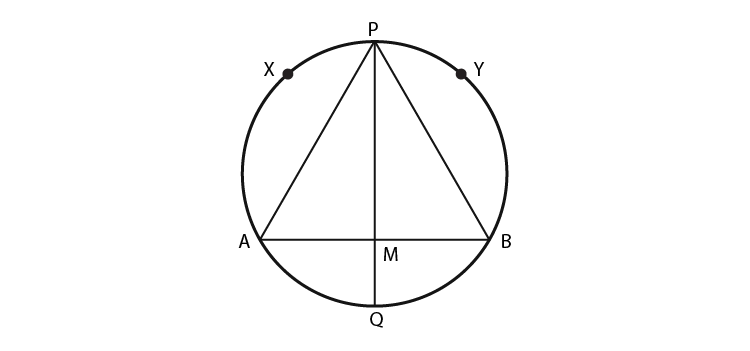According to the question,

We have,

PQ is the perpendicular bisect of AB,

So, we get,

AM = BM …eq.(1)

In △APM and △BPM,

From eq.(1),

AM = BM

∠AMP = ∠BMP = 90o

PM = PM [Common side]

Therefore, △APM ≅ △BPM [By SAS congruence rule]

So, AP = BP [CPCT]

Hence, arc PXA ≅ Arc PYB

Therefore, if two chords of a circle are equal, then their corresponding arcs are congruent.

3. A, B and C are three points on a circle. Prove that the perpendicular bisectors of AB, BC and CA are concurrent.

Solution:

According to the question,

Three non-collinear points A, B and C are on a circle.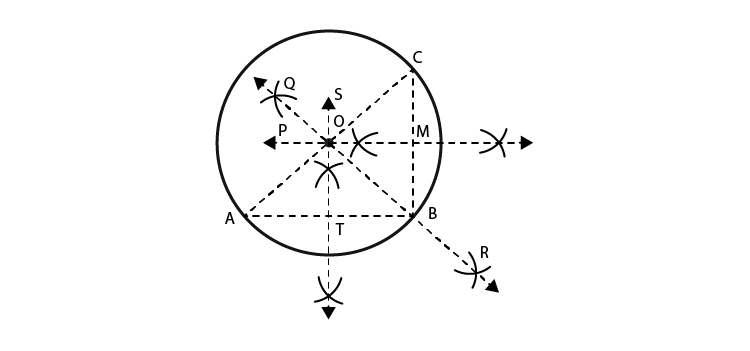To prove: Perpendicular bisectors of AB, BC and CA are concurrent.

Construction: Join AB, BC and CA.

Draw:

ST, perpendicular bisector of AB,

PM, perpendicular bisector of BC

And, QR perpendicular bisector of CA

As point A, B and C are not collinear, ST, PM and QR are not parallel and will intersect.

Proof:

O lies on ST, the ⊥ bisector of AB

OA = OB … (1)

Similarly, O lies on PM, the ⊥ bisector of BC

OB = OC … (2)

And, O lies on QR, the ⊥ bisector of CA

OC = OA … (3)

From (1), (2) and (3),

OA = OB = OC

Let OA = OB = OC = r

Draw circle, with centre O and radius r, passing through A, B and C.

Hence, O is the only point equidistance from A, B and C.

Therefore, the perpendicular bisectors of AB, BC and CA are concurrent.

4. AB and AC are two equal chords of a circle. Prove that the bisector of the angle BAC passes through the centre of the circle.

Solution: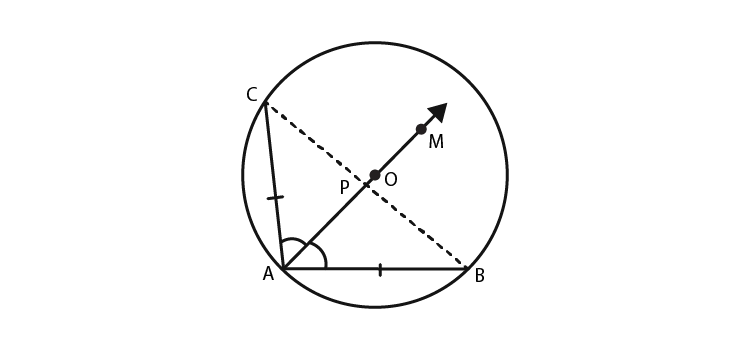According to the question,

We have,

AB and AC are two chords which are equal with centre O.

AM is the bisector of ∠BAC.

To prove: AM passes through O.

Construction: Join BC.

Let AM intersect BC at P.

Proof: In DBAP and DCAP

AB = AC [Given]

∠BAP = ∠CAP [Given]

And AP = BP [Common side]

△BAP ≅ △CAP [By SAS]

∠BPA = ∠CPA [CPCT]

We know that,

CP = PB

But, since ∠BPA and ∠CPA are linear pair angles,

We have,

∠BPA+∠CPA =180o

∠BPA = ∠CPA = 90o

Then,

AP is the perpendicular bisector of the chord BC, which will pass through the centre O on being produced.

Therefore, AM passes through O.

5. If a line segment joining mid-points of two chords of a circle passes through the centre of the circle, prove that the two chords are parallel.

Solution:

Consider AB and CD to be the chords of the circle with centre O.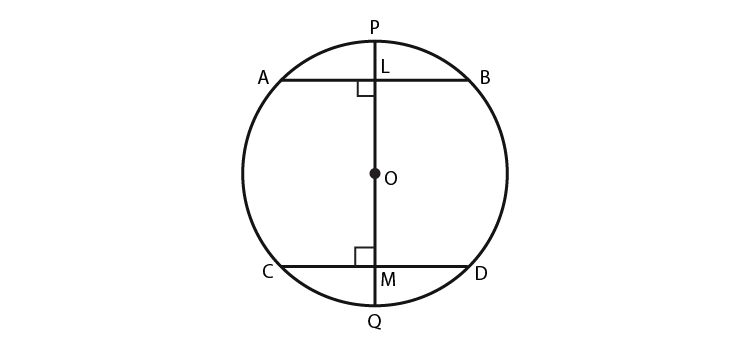Let L be the midpoint of AB.

Let M be the midpoint of CD.

Let PQ be the line passing through these midpoints and the centre of the circle.

Then, PQ is the diameter of the circle.

We know that,

Line joining centre to the midpoint of a chord is always perpendicular to the chord.

Since M is the midpoint of CD,

We have, OM ⊥ CD

⇒ OMD = 90°

Similarly, L is the midpoint of AB,

OL ⊥ AB

⇒ OLA = 90°

But, we know,

∠OLA and ∠OMD are alternate angles.

So, AB ∥ CD.

Hence, proved.

6. ABCD is such a quadrilateral that A is the centre of the circle passing through B, C and D. Prove that ∠CBD + ∠CDB = ½ ∠BAD

Solution:

According to the question,

We have,

A quadrilateral ABCD such that A is the centre of the circle passing through B, C and D.

Construction:

Join CA and BD.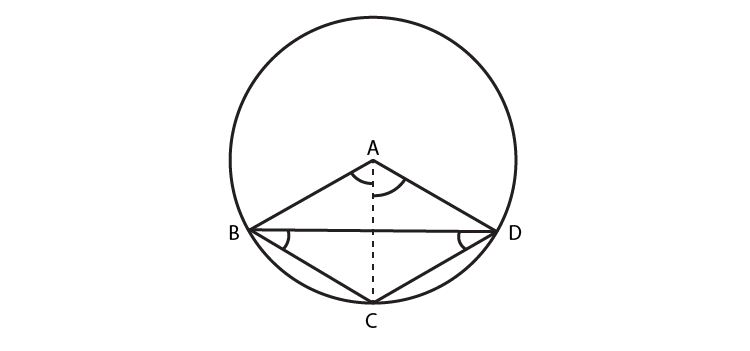We know that,

In a circle, angle subtended by an arc at the centre is twice the angle subtended by it at any other point in the remaining part of the circle

So,

The arc DC subtends ∠DAC at the centre and ∠CAB at point B in the remaining part of the circle,

We get,

∠DAC = 2∠CBD …(1)

Similarly,

The arc BC subtends ∠CAB at the centre and ∠CDB at point D in the remaining part of the circle,

We get,

∠CAB = 2∠CDB …(2)

From equations (1) and (2),

We have:

DAC + ∠CAB = 2∠CDB + 2∠CBD

⇒ ∠BAD = 2(∠CDB + ∠CBD)

⇒ (∠CDB + ∠CBD) = ½ (∠BAD)

7. O is the circumcentre of the triangle ABC, and D is the mid-point of the base BC. Prove that ∠BOD = ∠A.

Solution:

According to the question,

We have,

O is the circumcenter of the triangle ABC and D is the midpoint of BC.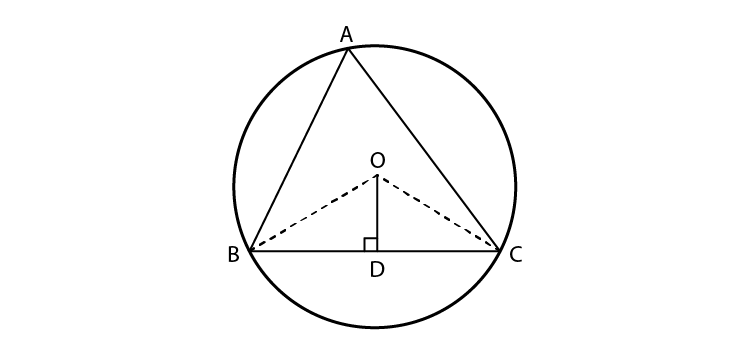To prove: ∠BOD = ∠A

Construction: Join OB and OC.

In ΔOBD and ΔCD:

OD = OD (common)

DB = DC (D is the midpoint of BC)

OB = OC (radius of the circle)

By SSS congruence rule,

We get,

ΔOBD ≅ ΔOCD.

∠BOD = ∠COD (By CPCT)

Let ∠BOD = ∠COD = x

We know that,

Angle subtended by an arc at the centre of the circle is twice the angle subtended by it at any other point in the remaining part of the circle.

So, we have,

2∠BAC = ∠BOC

⇒ 2∠BAC = ∠BOD + ∠DOC

⇒ 2∠BAC = x + x

⇒ 2∠BAC = 2x

⇒ ∠BAC = x

⇒ ∠BAC = ∠BOD

Hence, proved.

8. On a common hypotenuse AB, two right triangles ACB and ADB are situated on opposite sides. Prove that ∠BAC = ∠BDC.

Solution:

According to the question,

We have,

ACB and ADB are two right triangles.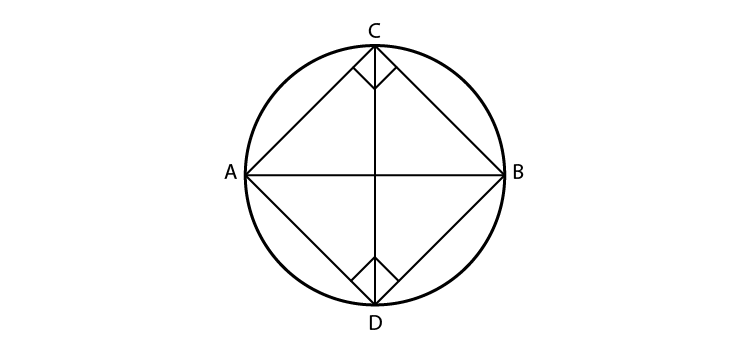To Prove: ∠BAC = ∠BDC

We know that,

ACB and ADB are right angled triangles,

Then,

∠C + ∠D = 90° + 90°

∠C + ∠D = 180°

Therefore ADBC is a cyclic quadrilateral as sum of opposite angles of a cyclic quadrilateral = 180°

We also have,

∠BAC and ∠BDC lie in the same segment BC and angles in the same segment of a circle are equal.

∴ ∠BAC = ∠BDC.

Hence Proved.

9. Two chords AB and AC of a circle subtend angles equal to 90º and 150º, respectively at the centre. Find ∠BAC, if AB and AC lie on the opposite sides of the centre.

Solution:

According to the question,

We have,

In ΔAOB,

OA = OB (radius of the circle)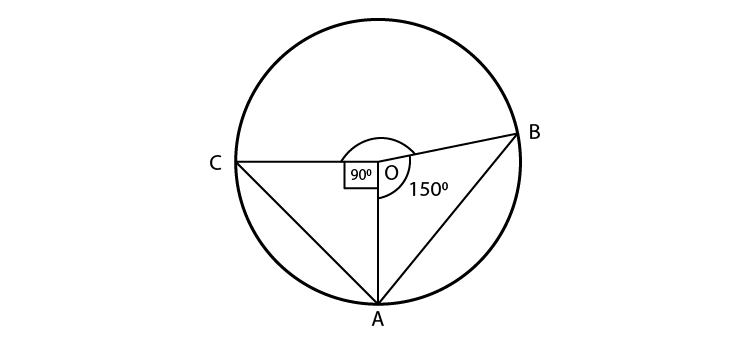Since angle opposite to equal sides are equal, we get,

∠OBA = ∠OAB

We know that,

According to angle sum property, sum of all angles of a triangle = 180o

Using the angle sum property in ΔAOB, we get,

∠OAB + ∠AOB +∠OBA = 180°

⇒ ∠OAB +90° + ∠OAB = 180°

⇒ 2∠OAB = 180° – 90°

⇒ 2∠OAB = 90°

⇒ ∠OAB = 45°

Now, in ΔAOC,

OA = OC (radius of the circle)

Since, angle opposite to equal sides are equal

∴ ∠OCA = ∠OAC

Using the angle sum property in ΔAOB, sum of all angles of the triangle is 180°, we have:

∠OAC + ∠AOC +∠OCA = 180°

⇒ ∠OAC +150° + ∠OAC = 180°

⇒ 2∠OAC = 180° – 150°

⇒ 2∠OAC = 30°

⇒ ∠OAC = 15°

Now, ∠BAC = ∠OAB + ∠OAC

= 45° + 15°

= 60°

∴ ∠BAC = 60°

10. If BM and CN are the perpendiculars drawn on the sides AC and AB of the triangle ABC, prove that the points B, C, M and N are concyclic.

Solution: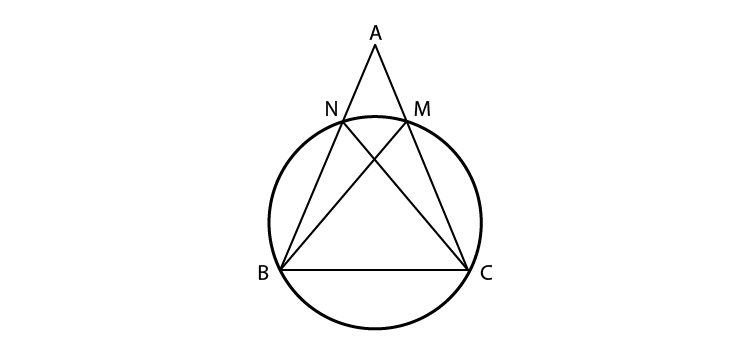According to the question,

BM and CN are the perpendiculars drawn on the sides AC and AB of the triangle ABC.

So, we have,

∠BMC = ∠BNC = 90o

We know that,

If a line segment joining two points subtends equal angles on the same side of the line containing the segment, then the four points are concyclic.

Considering the question,

Since BC joins the two points, B and C, subtending equal angles, ∠BMC and ∠BNC, at M and N on the same side BC containing the segment, then B, C, M and N are concyclic.

Hence, we get that,

B, C, M and N are concyclic.

Exercise 10.4 Page No: 64

1. If two equal chords of a circle intersect, prove that the parts of one chord are separately equal to the parts of the other chord.

Solution:

According to the question,

AB and CD are two equal chords of a circle with centre O, intersecting each other at M.

To prove:

(i) MB = MC and

(ii) AM = MD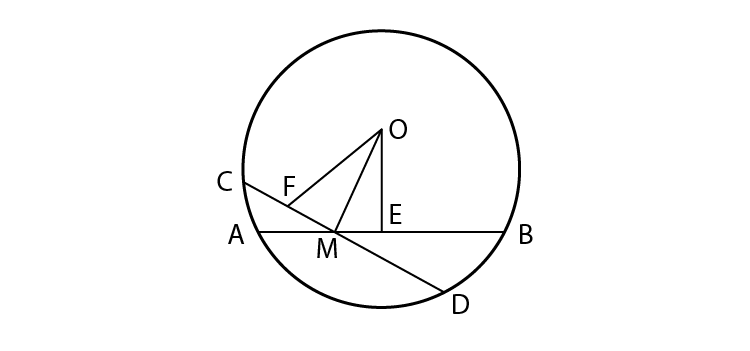Proof:

AB is a chord and OE ⊥ to AB from the centre O,

Since, perpendicular from the centre to a chord bisect the chord

We get,

AE = ½ AB

Similarly,

FD = ½ CD

It is given that,

AB = CD⇒ ½ AB = ½ CD

So, AE = FD … (1)

Since equal chords are equidistance from the centre,

And AB = CD

So, OE = OF

Now, as proved, in right triangles MOE and MOF,

hyp. OE = hyp. OF [Common side]

OM = OM

ΔMOE ≅ ΔMOF

ME = MF … (2)

Subtracting equations (2) from (1), we get

AE – ME = FD – MF

⇒ AM = MD [Proved part (ii)]

Again, AB = CD [Given]

And AM = MD [Proved]

AB – AM = CD – MD [Equals subtracted from equal]

Hence, MB = MC [Proved part (i)]

2. If non-parallel sides of a trapezium are equal, prove that it is cyclic.

Solution:According to the question,

We have,

ABCD is a trapezium in which AD||BC

Non-parallel sides AB and DC of the trapezium ABCD are equal i.e.,

AB = DC.

To prove: Trapezium ABCD is cyclic.

Construction: Draw AM and DN such that they are perpendicular on BC.

Proof: In right triangles AMB and DNC,

∠AMB = ∠DNC = 90o

AB = DC [Given]

Since perpendicular distance between two parallel lines are same,

AM = DN

ΔAMB ≅ ΔDNC [By RHS congruence rule]

∠B = ∠C [CPCT]

And ∠1 = ∠2

= ∠2 + 90

= ∠CDA

∠B +∠B +∠CDA+∠CDA = 360

2(∠B +∠CDA) = 360

∠B +∠CDA =180

We know that,

If any pair of opposite angles of a quadrilateral is 180o, then the quadrilateral is cyclic.

Hence, the trapezium ABCD is cyclic.

3. If P, Q and R are the mid-points of the sides BC, CA and AB of a triangle and AD is the perpendicular from A on BC, prove that P, Q, R and D are concyclic.

Solution:

To prove: R, D, P and Q are concyclic.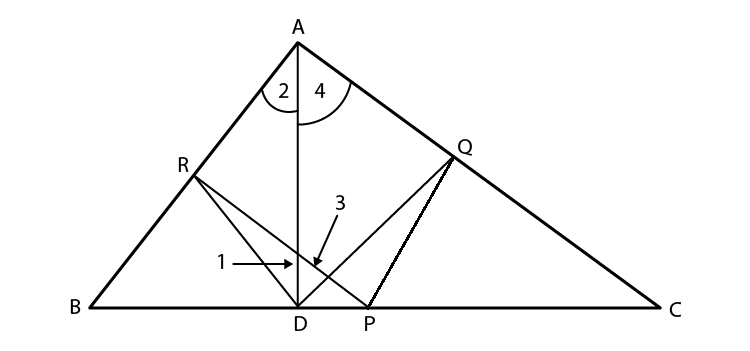Construction: Join RD, QD, PR and PQ.

RP joins the mid-point of AB, i.e., R, and the mid-point of BC, i.e., P.

Using midpoint theorem,

RP||AC

Similarly,

PQ||AB.

So, we get,

ARPQ is a parallelogram.

So, ∠RAQ = ∠RPQ [Opposite angles of a ||gm]…(1)

ABD is a right angled triangle and DR is a median,

RA = DR and ∠1 = ∠2 …(2)

Similarly ∠3 = ∠4 …(3)

We get,

∠1+ ∠3 = ∠2 +∠4

⇒ ∠RDQ = ∠RAQ

∠RPQ [Proved above]

Since ∠D and ∠P are subtended by RQ on the same side of it, we get the points R, D, P and Q concyclic.

Hence, R, D, P and Q are concyclic.

4. ABCD is a parallelogram. A circle through A, B is so drawn that it intersects AD at P and BC at Q. Prove that P, Q, C and D are concyclic.

Solution:

According to the question,

ABCD is a parallelogram.

A circle through A, B is so drawn that it intersects AD at P and BC at Q.

To prove: P, Q, C and D are concyclic.

Construction: Join PQ.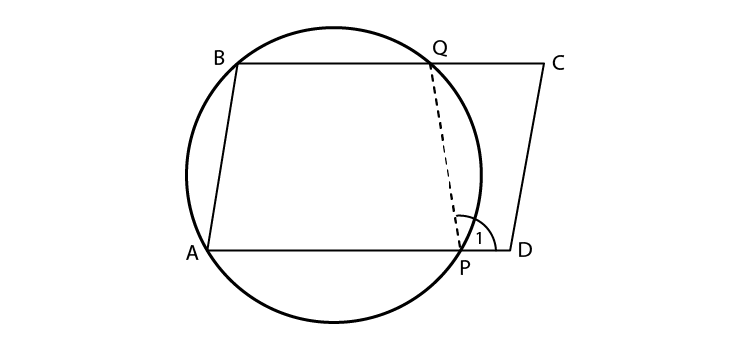Extend side AP of the cyclic quadrilateral APQB to D.

External angle, ∠1= interior opposite angle, ∠B

Since, BA||CD and BC cuts them

∠B +∠C =180o

Since, Sum of interior angles on the same side of the transversal = 180o

Or ∠1+∠C =180o

Hence, the points P, Q, C and D are concyclic.

5. Prove that angle bisector of any angle of a triangle and perpendicular bisector of the opposite side, if intersect, they will intersect on the circumcircle of the triangle.

Solution:

According to the question,

Triangle ABC and l is perpendicular bisector of BC.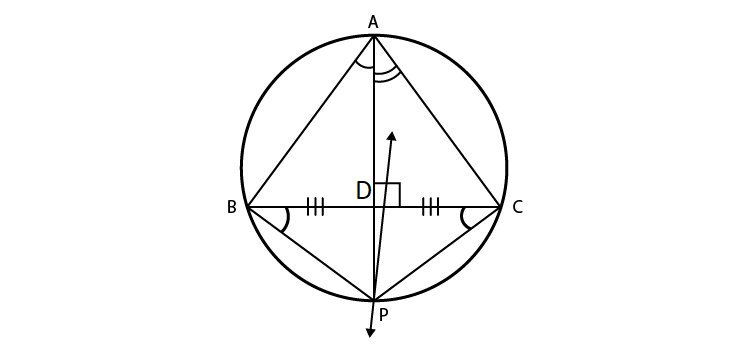To prove:

Angles bisector of ∠A and perpendicular bisector of BC intersect on the circumcircle of ΔABC.

Proof:

Let the angle bisector of ∠A intersect the circumcircle of ΔABC at D.

Construction: Join BP and CP.

Since, angles in the same segment are equal

We have, ∠BAP = ∠BCP

We know that,

AP is bisector of ∠A.

Then,

∠BAP = ∠BCP = ½ ∠A …(1)

Similarly,

We have,

∠PAC = ∠PBC = ½ ∠A …(2)

From equations (1) and (2),

We have

∠BCP = ∠PBC

We know that,

If the angles subtended by two Chords of a circle at the centre are equal, the chords are equal.

So,

BP = CP

Here, P is on the perpendicular bisector of BC.

Hence, the angle bisector of ∠A and the perpendicular bisector of BC intersect on the circumcircle of ΔABC.

Stay tuned to BYJU’S and get updated learning materials for all the chapters of Class 9 for all subjects. Also, download BYJU’S – The Learning App to get personalised video content and learn the concepts of Maths, such as circles and its fundamentals, in an easy way.

## Frequently Asked Questions on NCERT Exemplar Solutions for Class 9 Maths Chapter 10

Q1

### Explain the concept of Cyclic quadrilaterals covered in Chapter 10 of NCERT Exemplar Solutions for Class 9 Maths.

A cyclic quadrilateral is a quadrilateral which has all its four vertices lying on a circle. It is also sometimes called an inscribed quadrilateral. The circle, which consists of all the vertices of any polygon on its circumference, is known as the circumcircle or circumscribed circle. If all four vertices of a quadrilateral ABCD lie on the circumference of the circle, then ABCD is a cyclic quadrilateral. In other words, if any four points on the circumference of a circle are joined, they form the vertices of a cyclic quadrilateral. It can be visualised as a quadrilateral which is inscribed in a circle, i.e., all four vertices of the quadrilateral lie on the circumference of the circle.
Q2

### What is an arc of a circle as per Chapter 10 of NCERT Exemplar Solutions for Class 9 Maths?

The arc of a circle is defined as the part or segment of the circumference of a circle. A straight line that could be drawn by connecting the two ends of the arc is known as a chord of a circle. If the length of an arc is exactly half of the circle, it is known as a semicircular arc.
Q3

### Mention the topics and subtopics covered in Chapter 10 of NCERT Exemplar Solutions for Class 9 Maths.

The topics and subtopics covered in Chapter 10 of NCERT Exemplar Solutions for Class 9 Maths are listed below:
1. Circles and their related terms like arc, sector, chords, semicircle, segments, etc.
2. The angle subtended by chords of a circle at a point and theorems based on it
3. The perpendicular from the centre to a chord and theorems based on it
4. Circle through three points and its related theorems
5. Chords equidistant from the centre of a circle
6. Angle subtended by an arc of a circle
7. Angles in the same segment of a circle
8. Cyclic quadrilaterals and related theorems